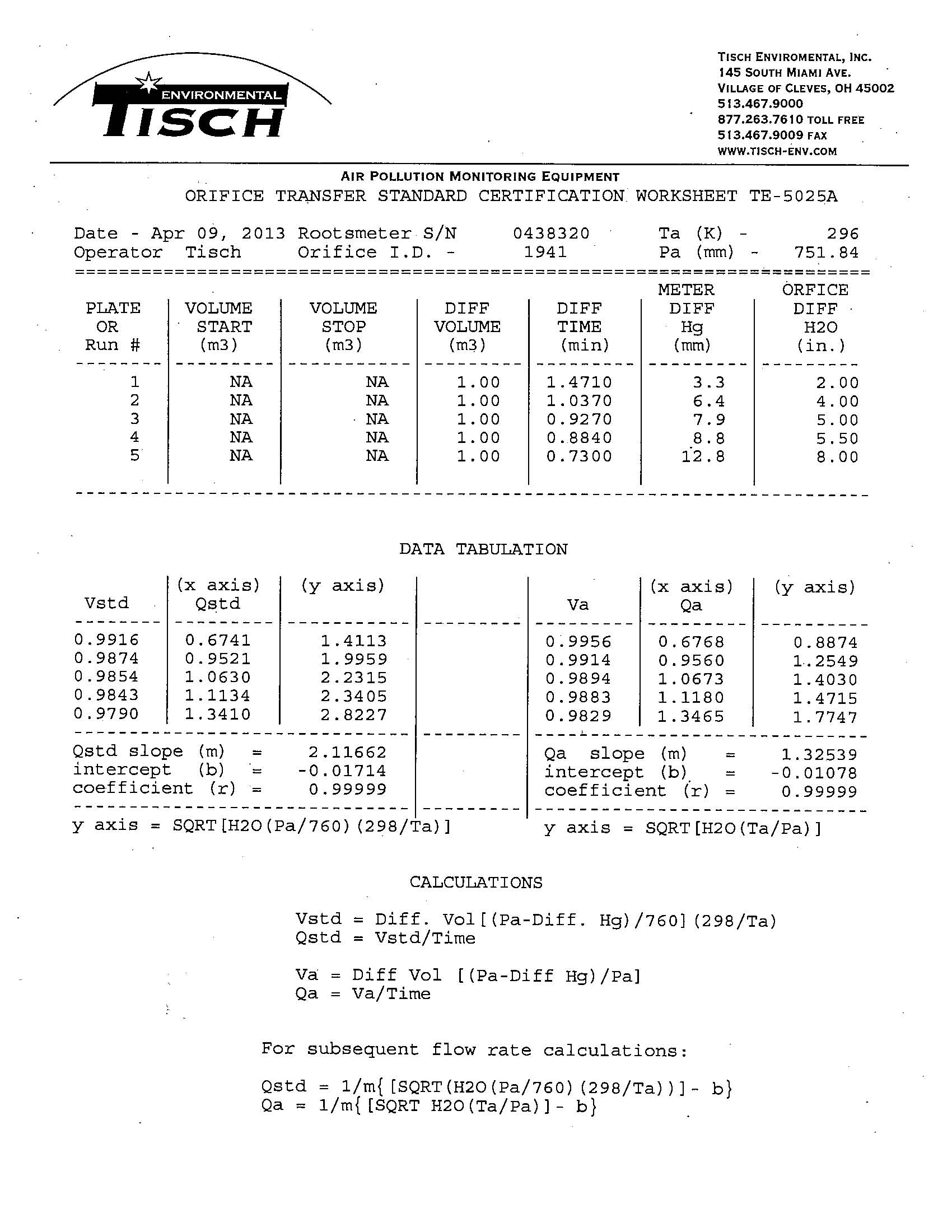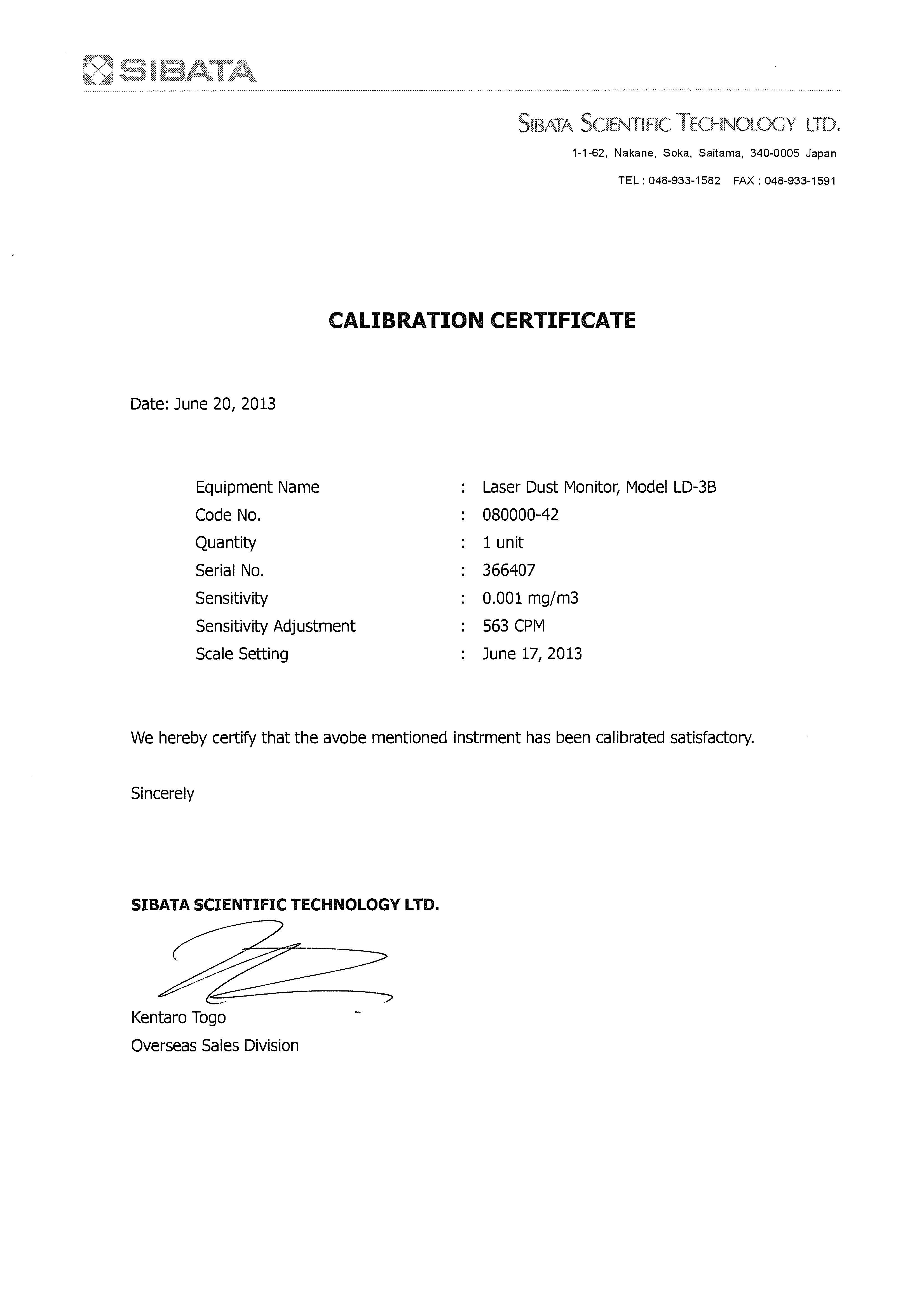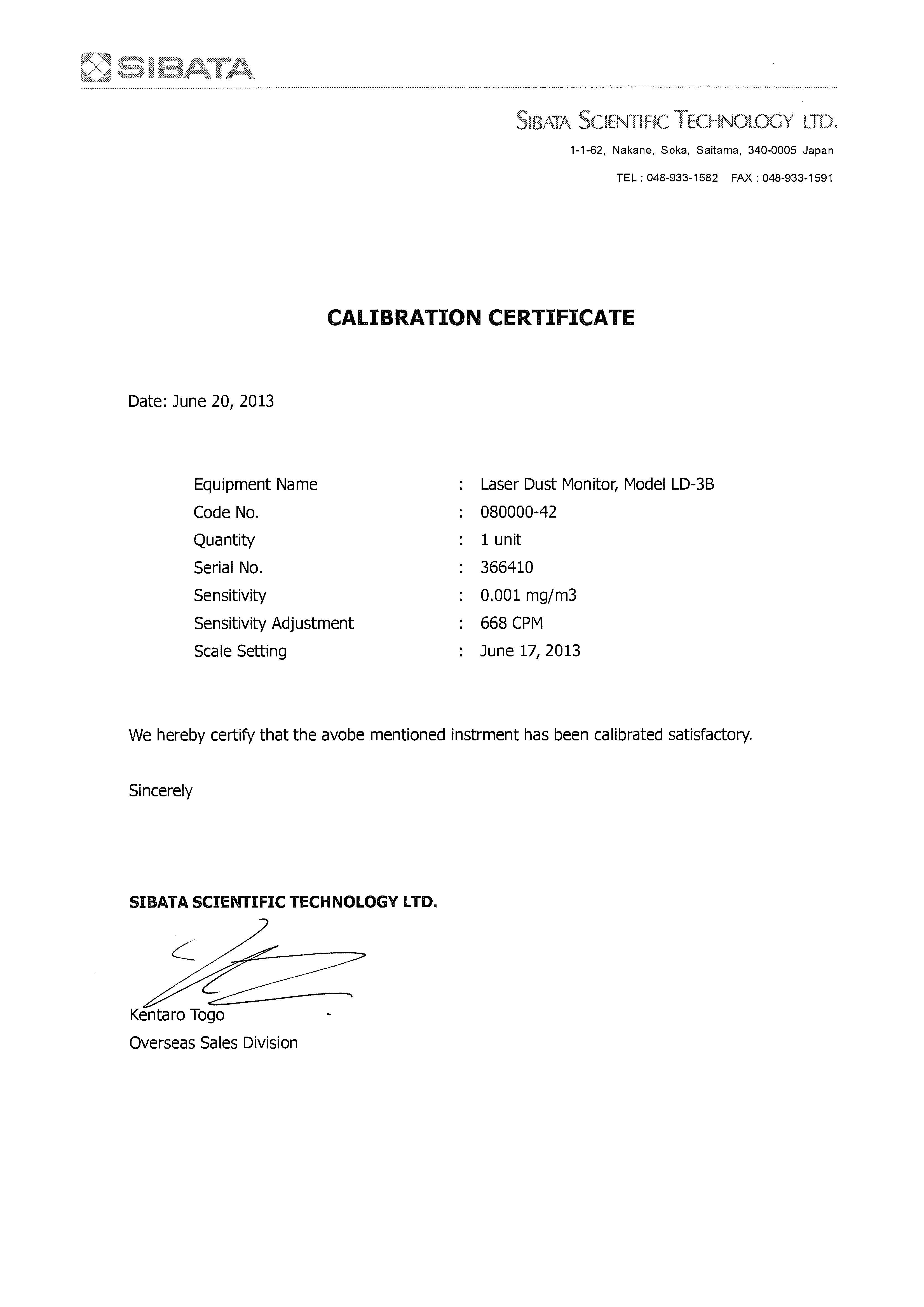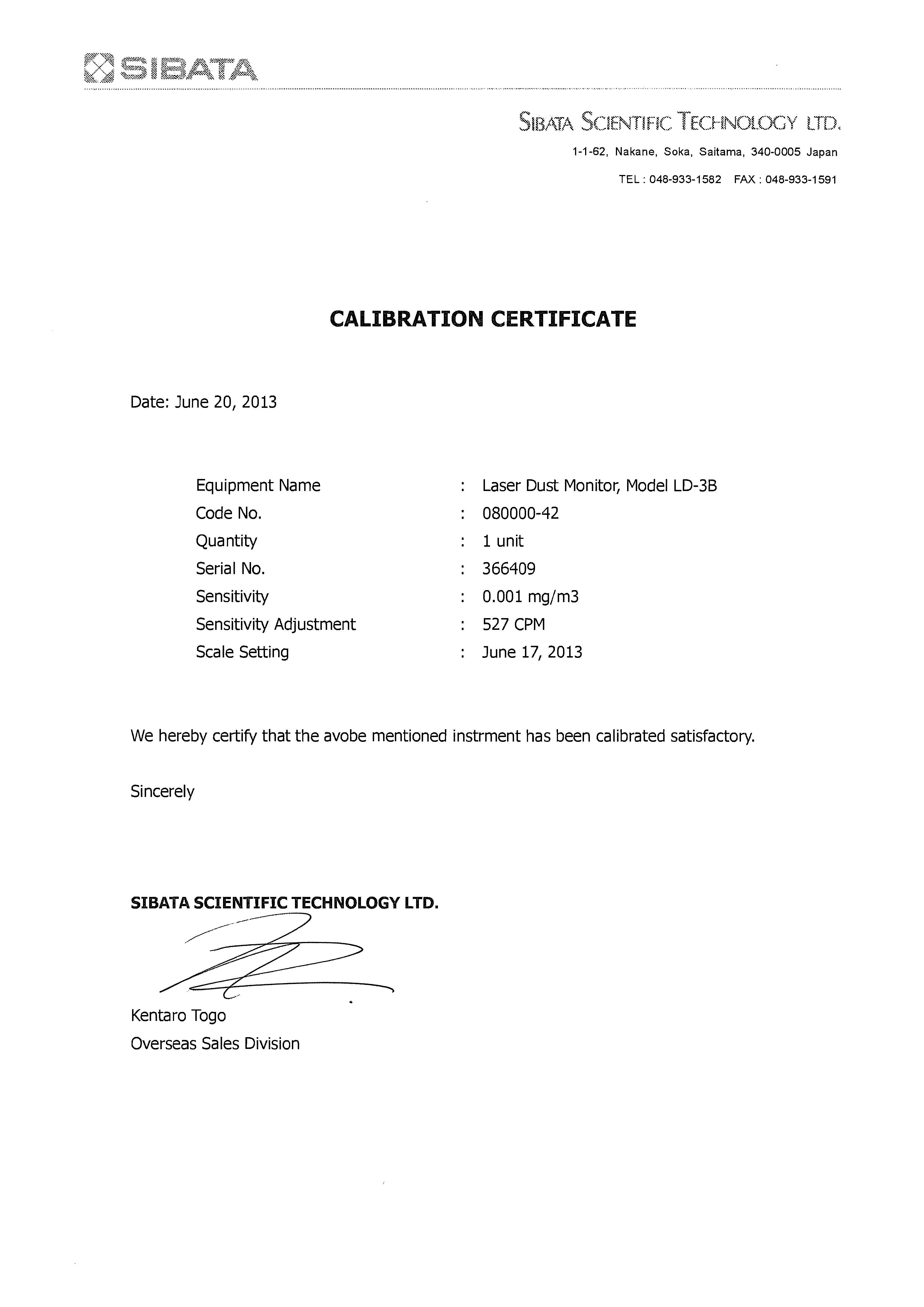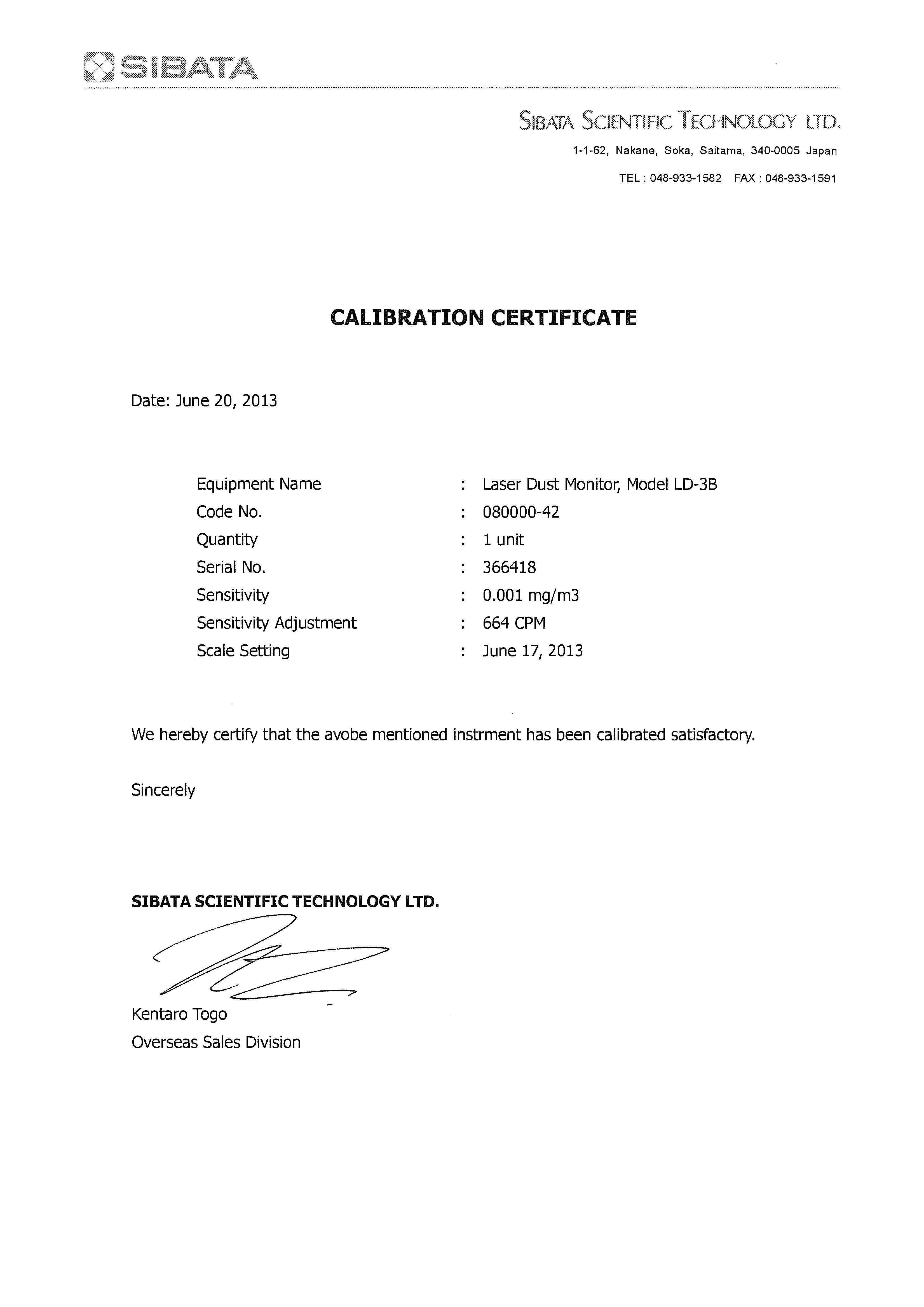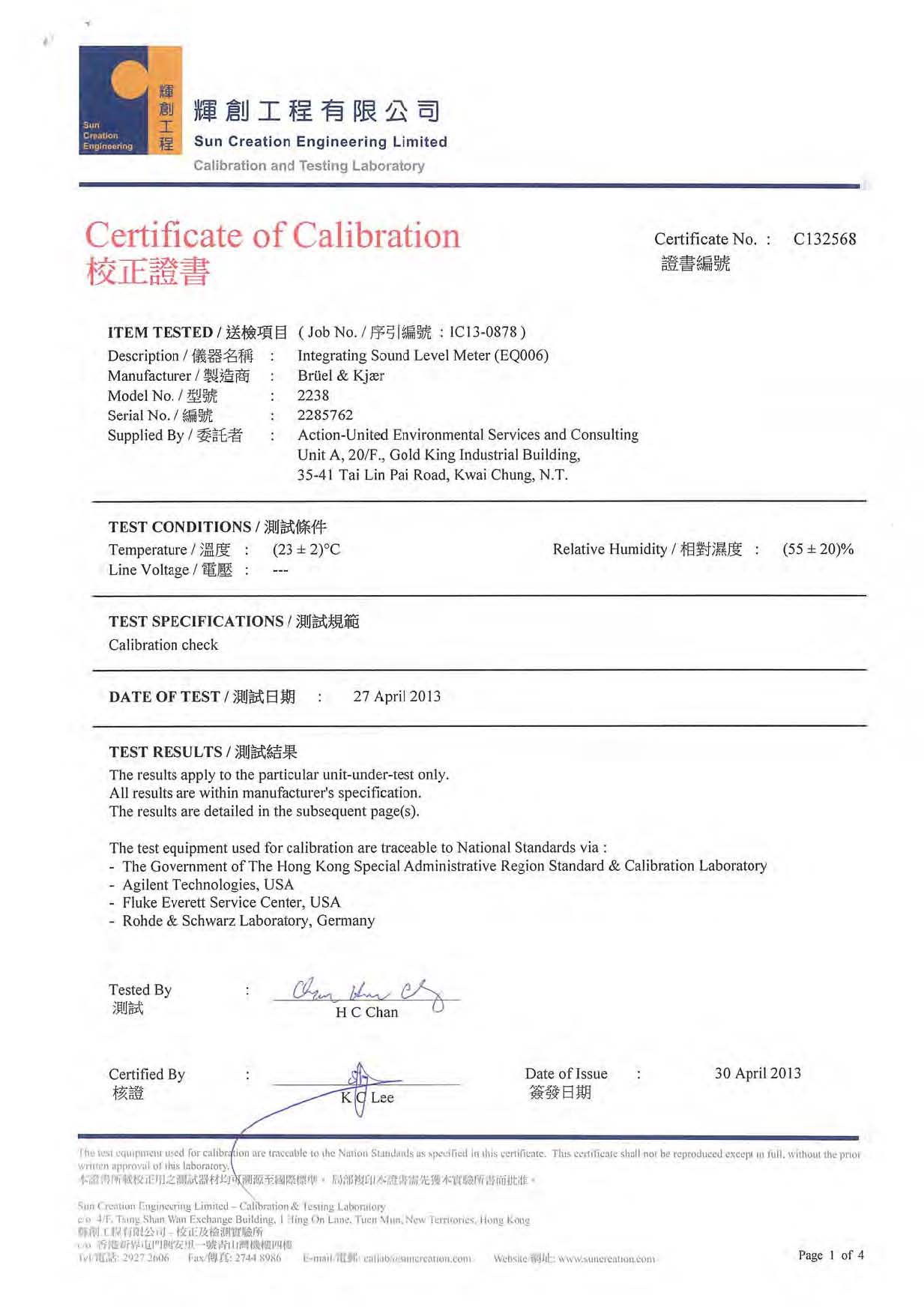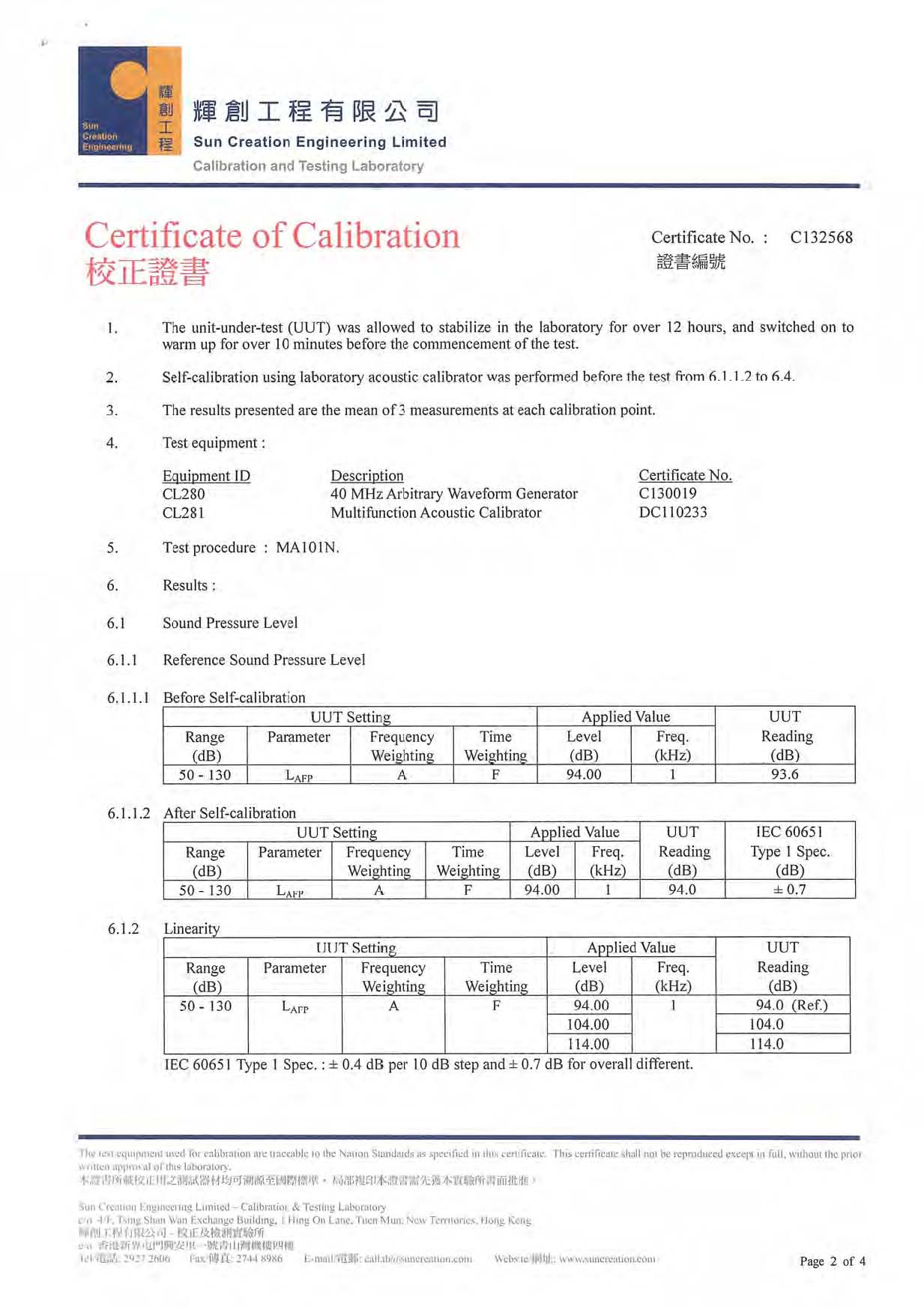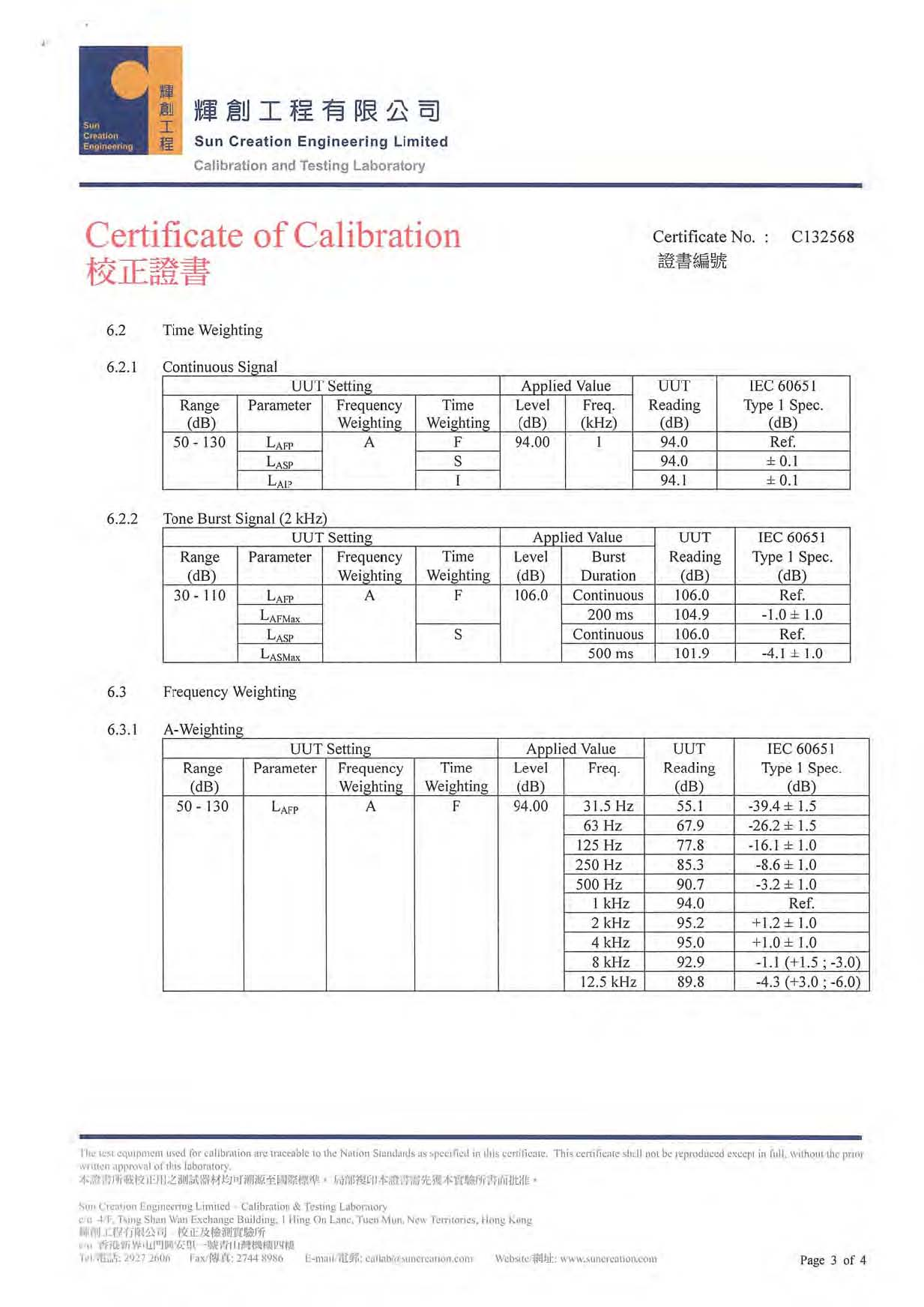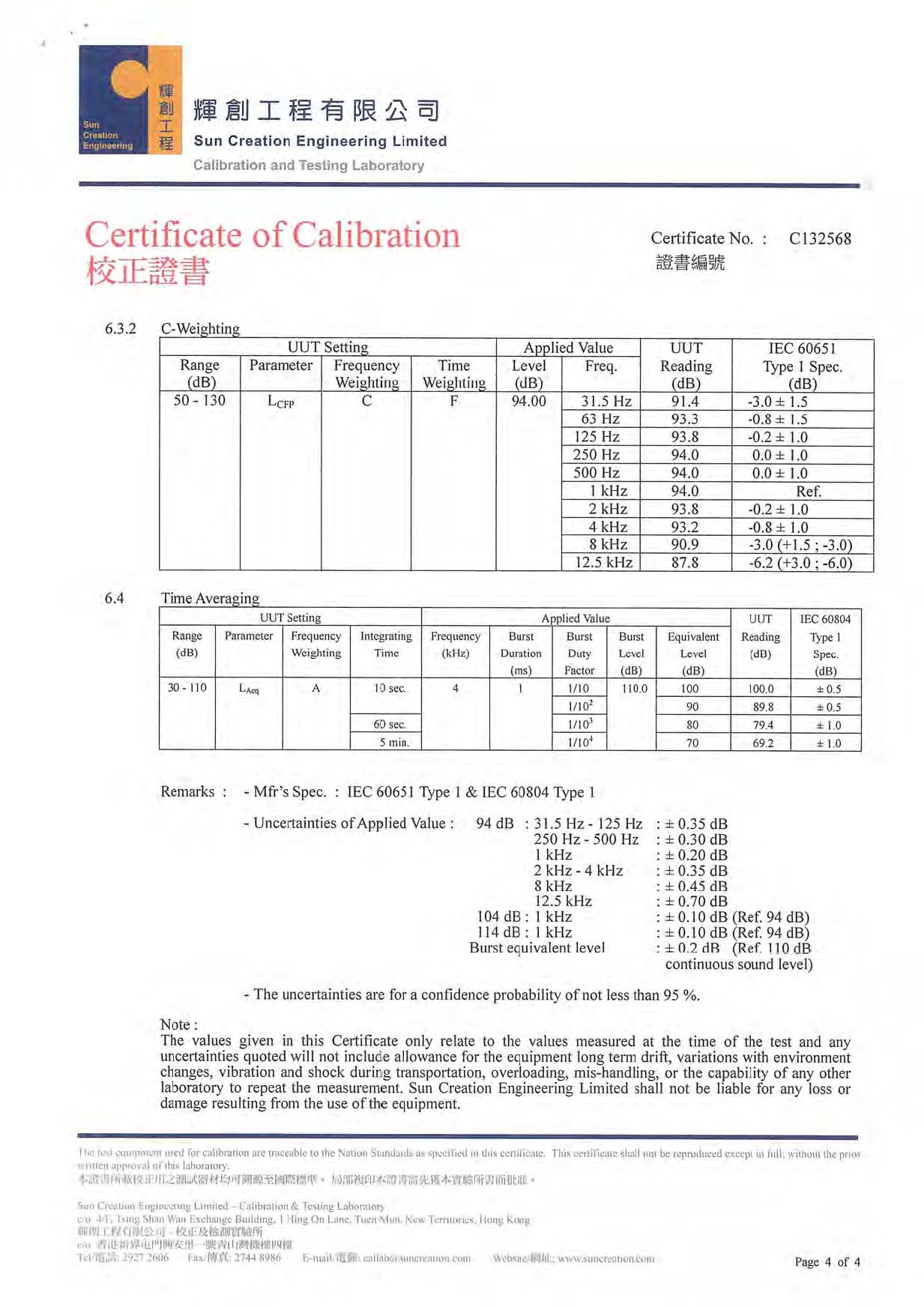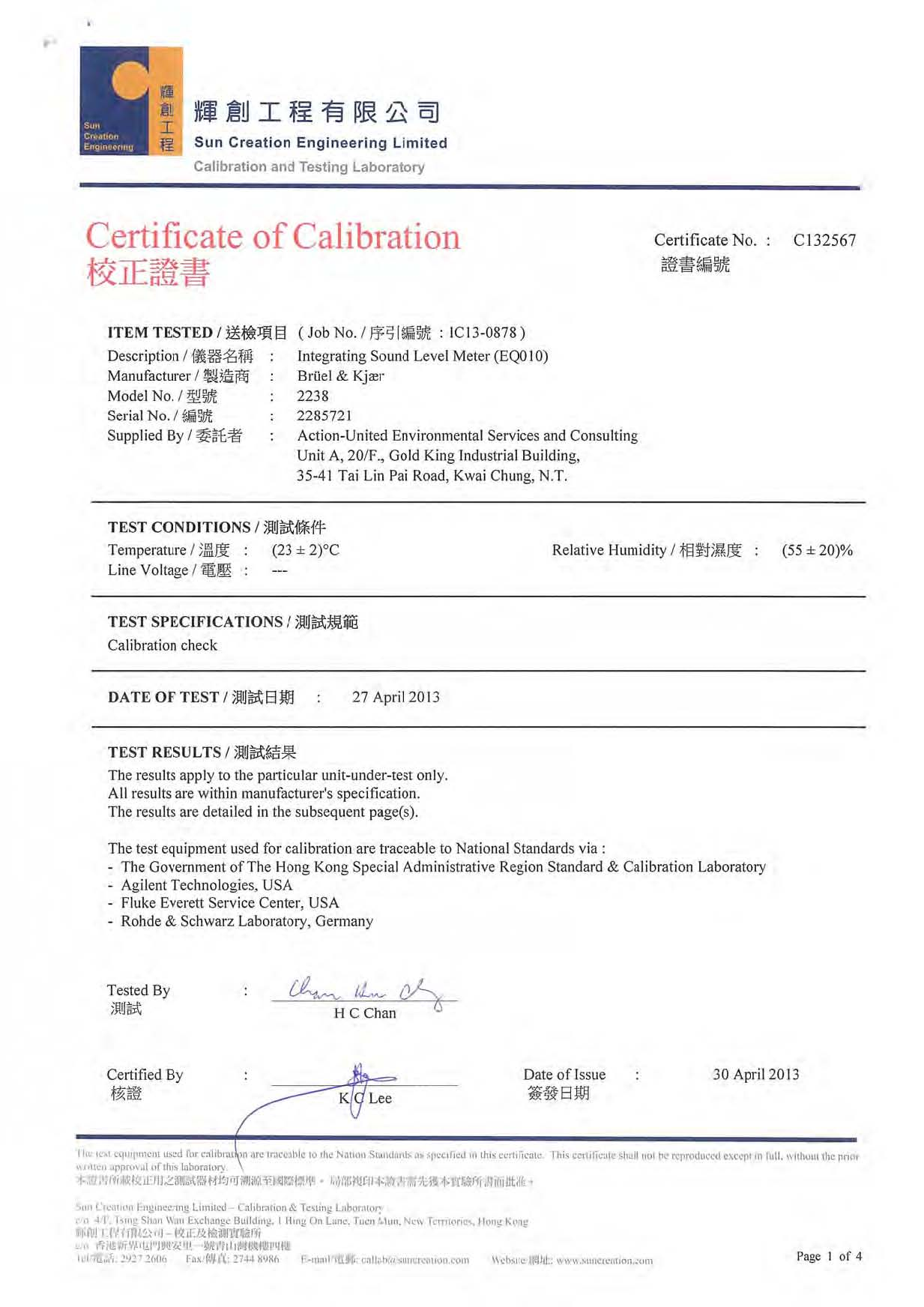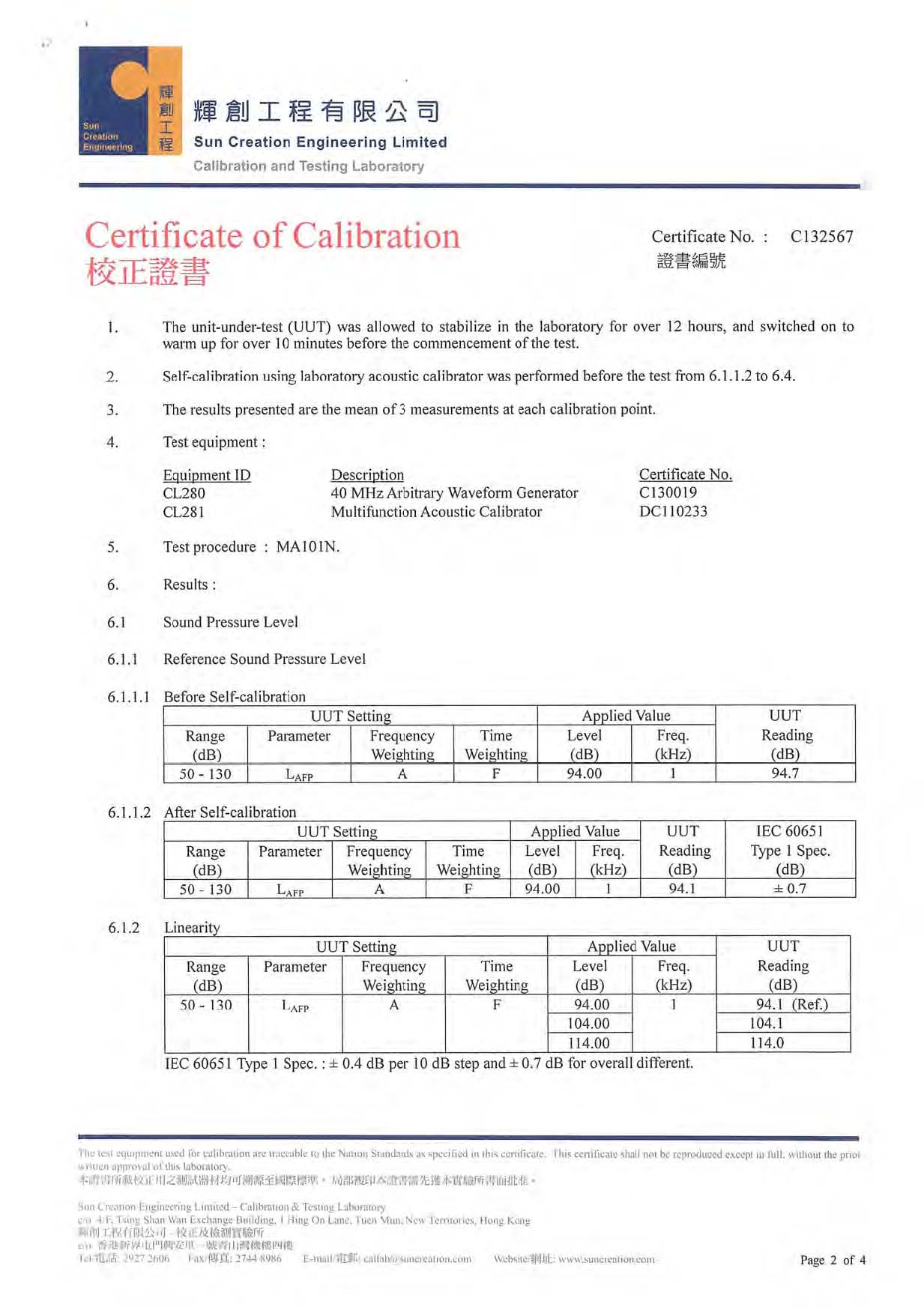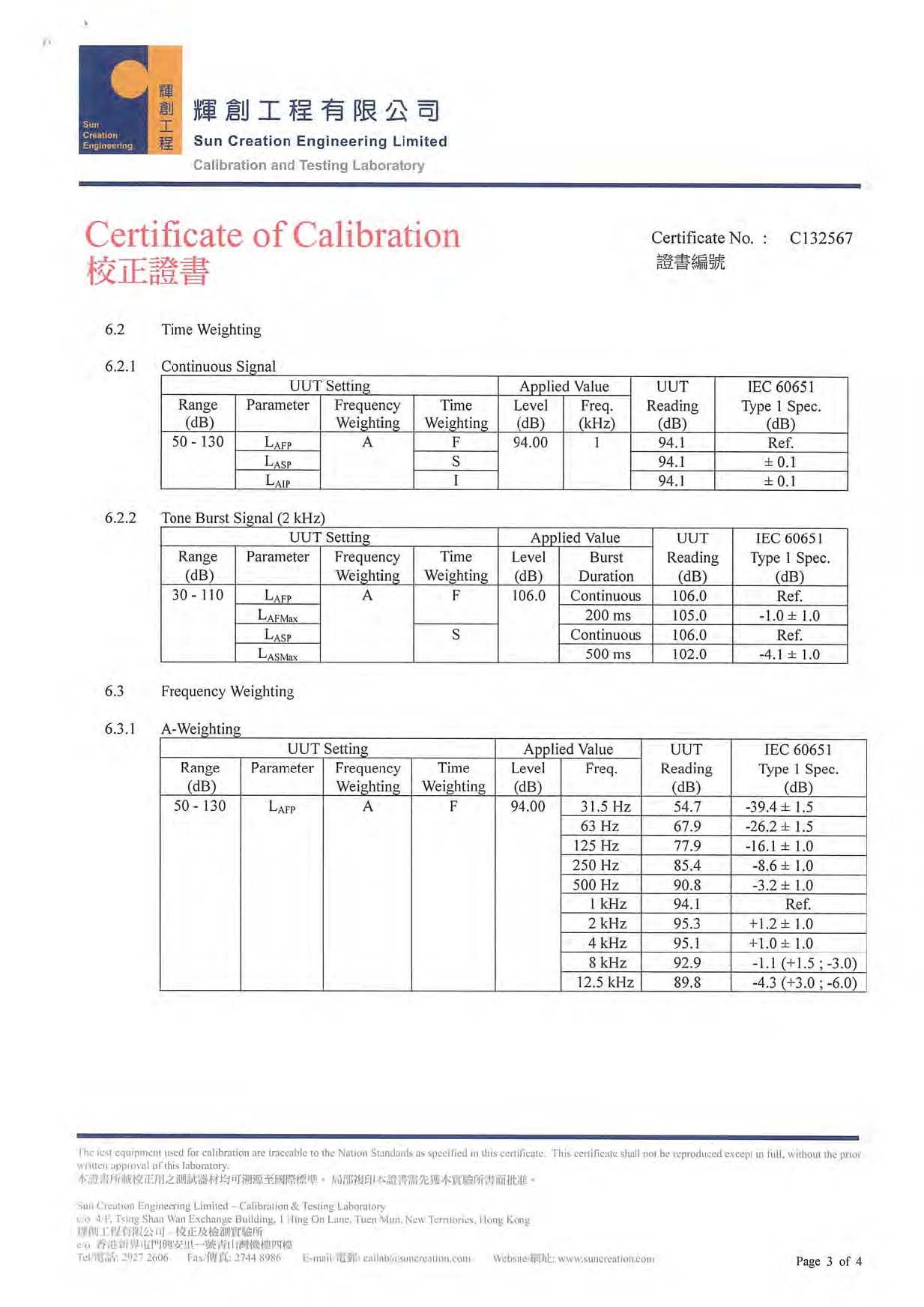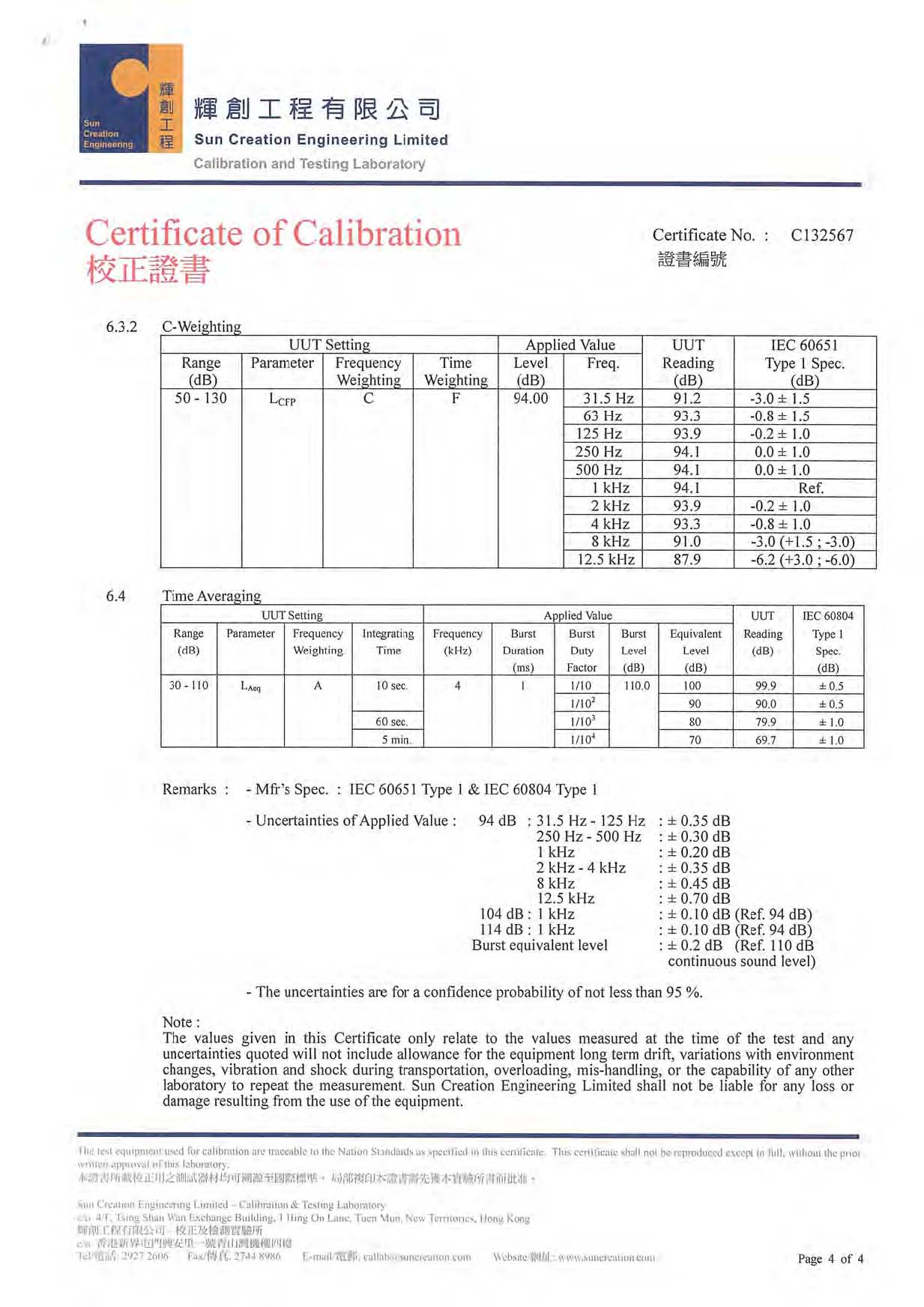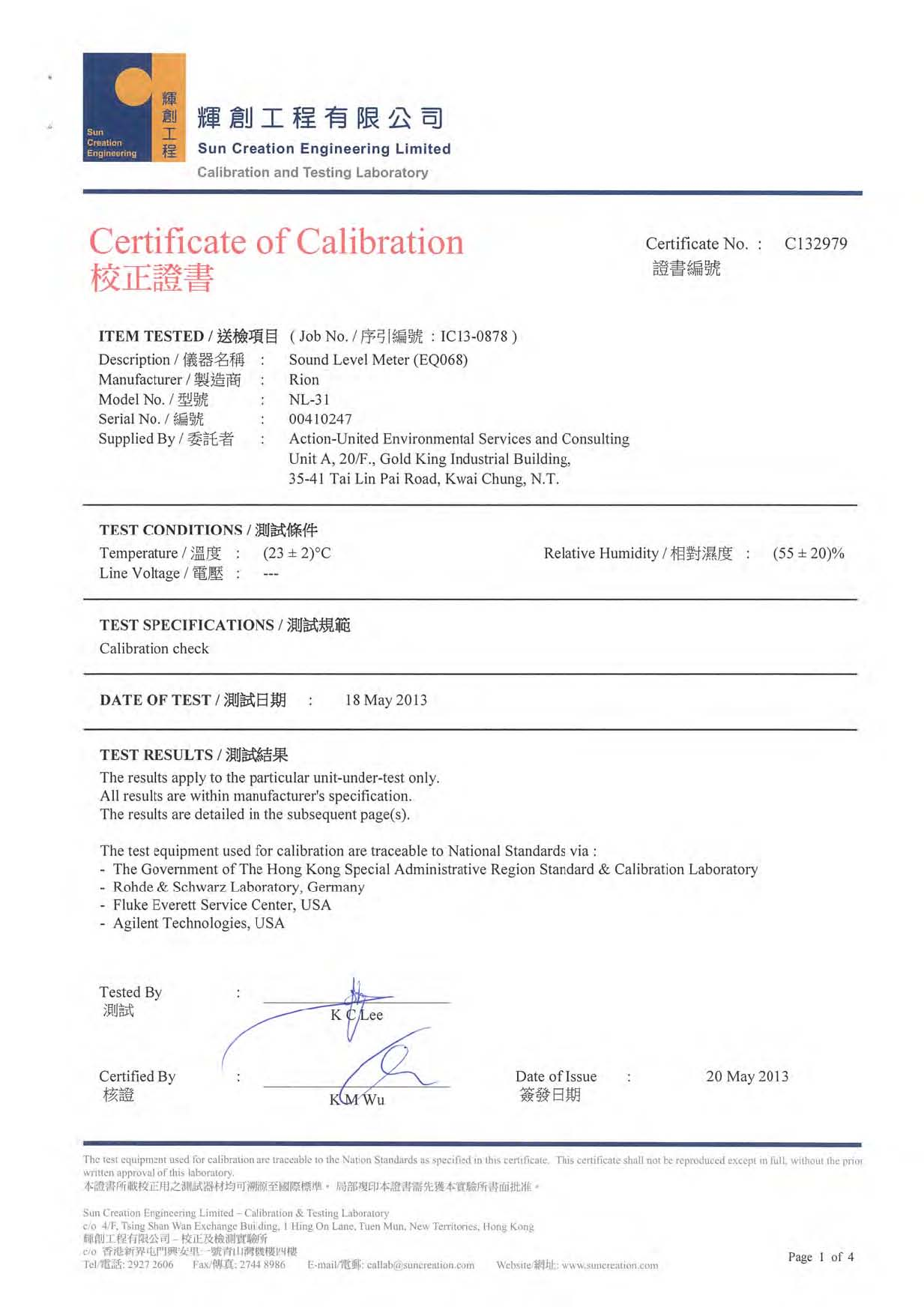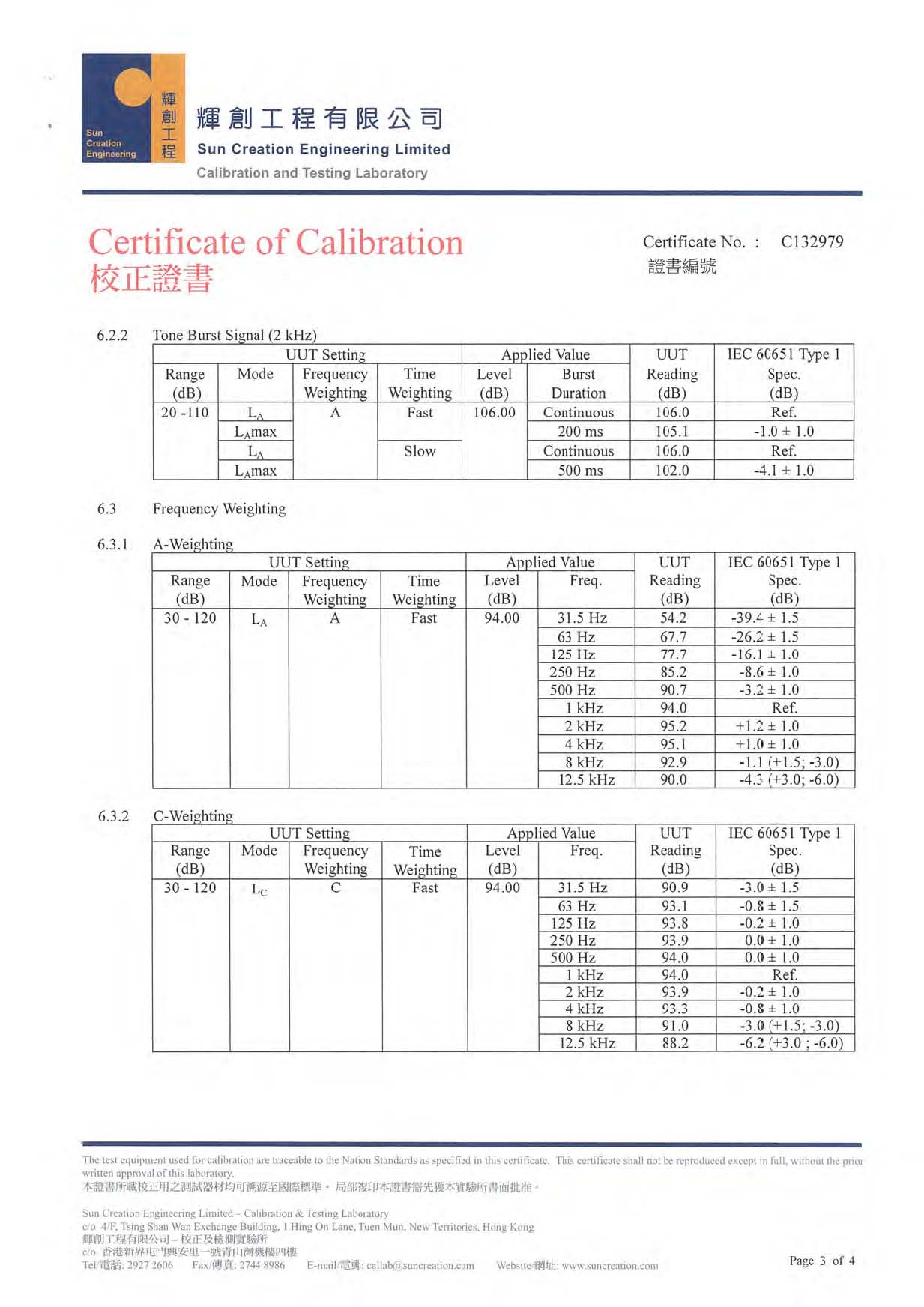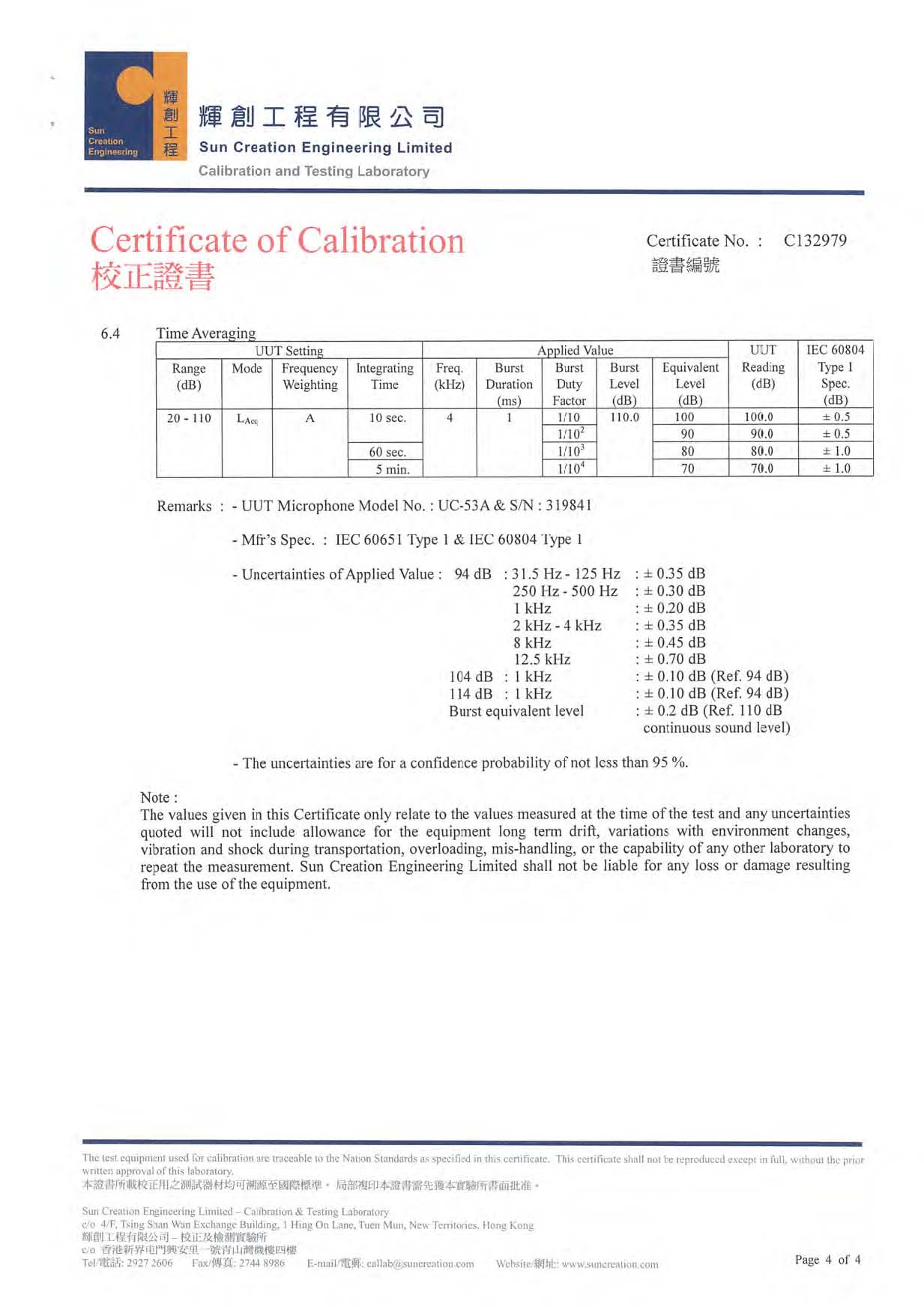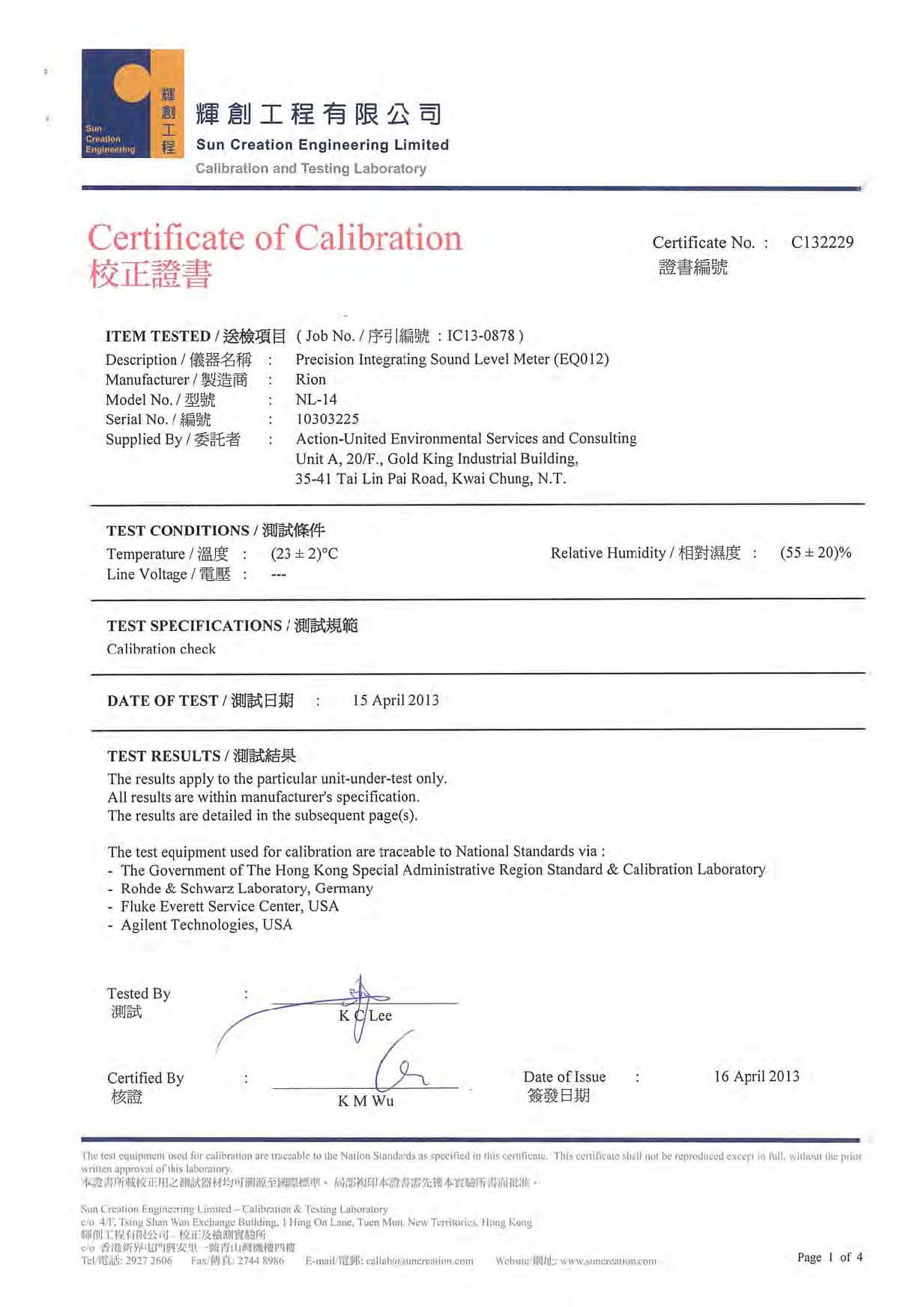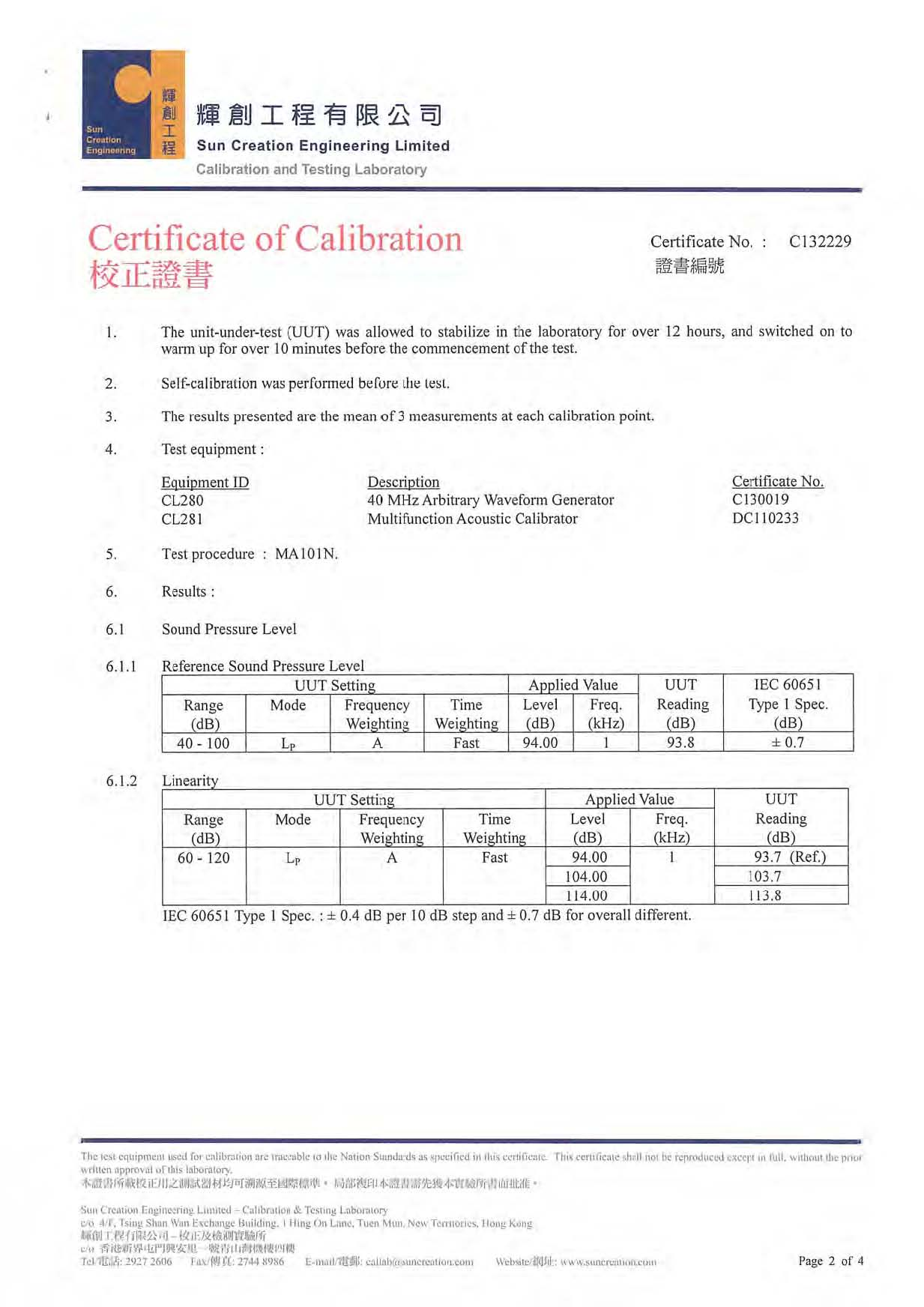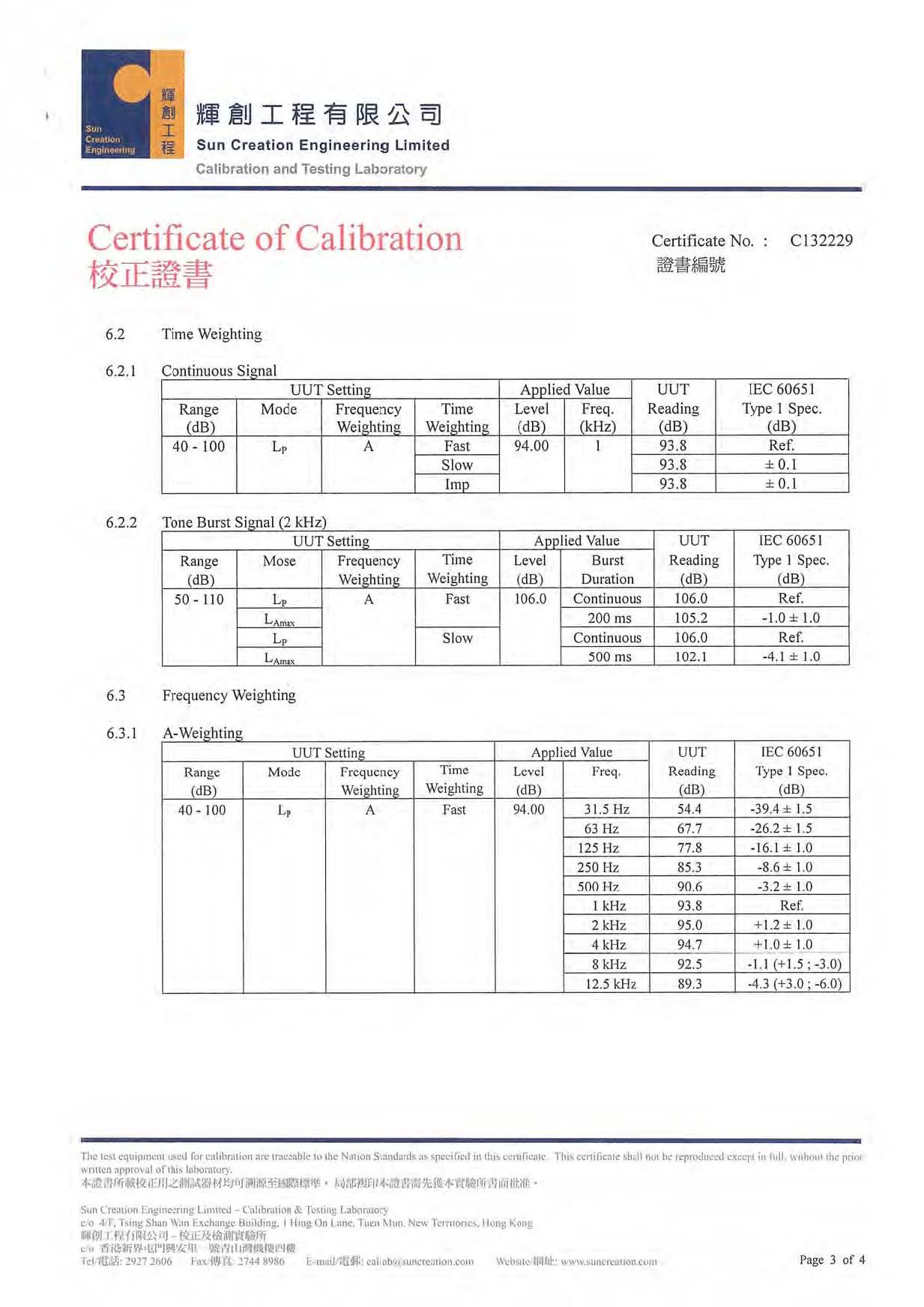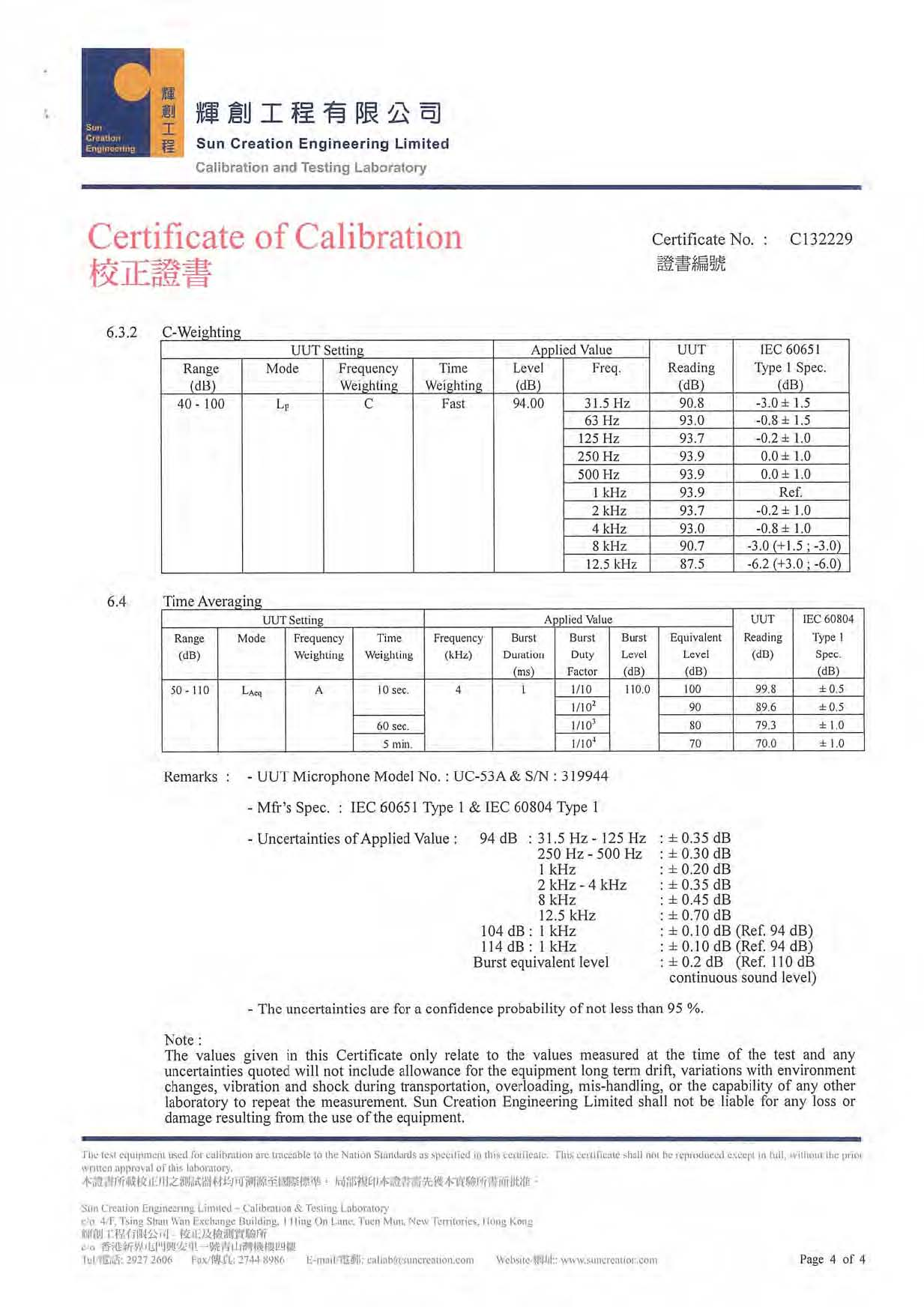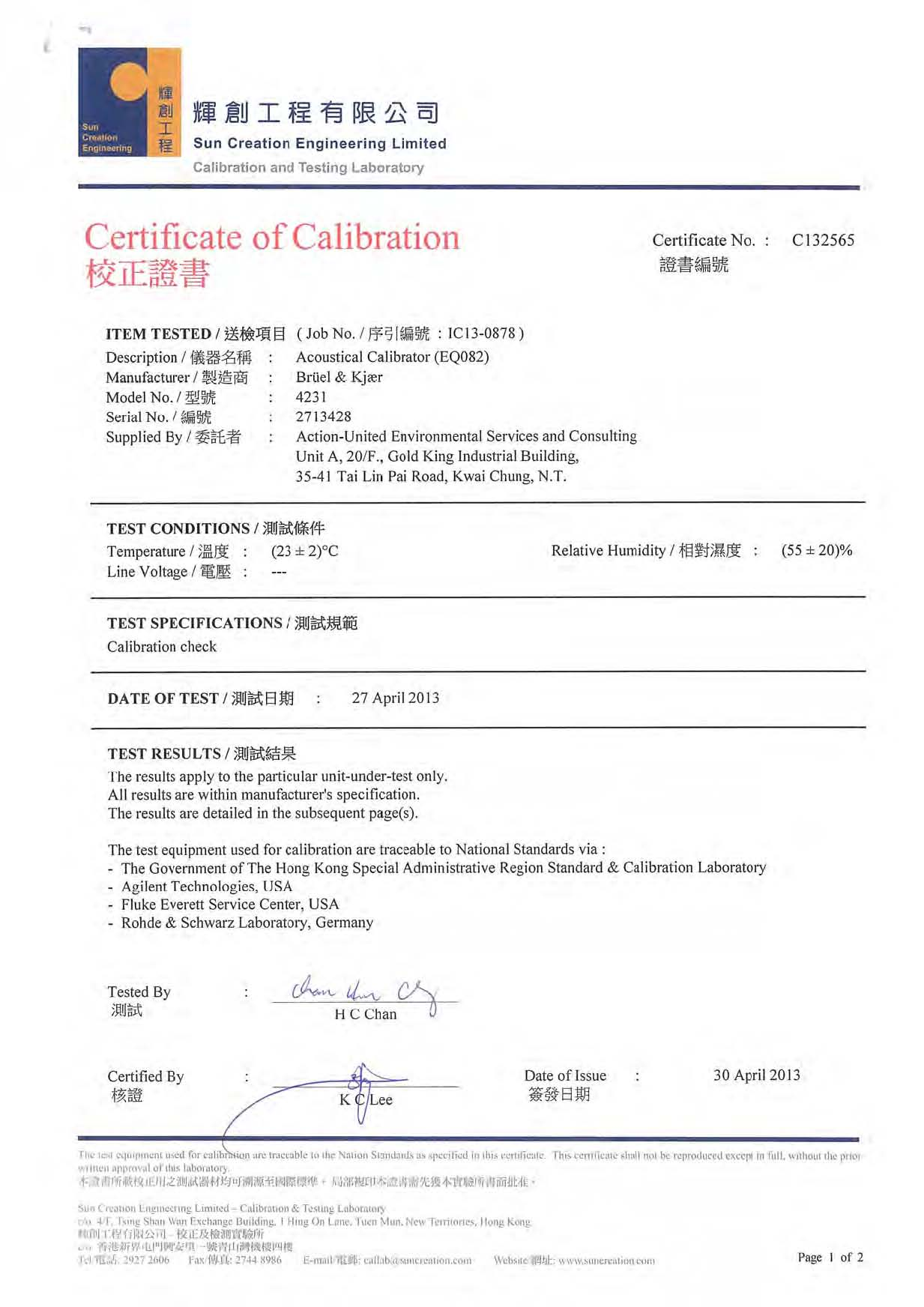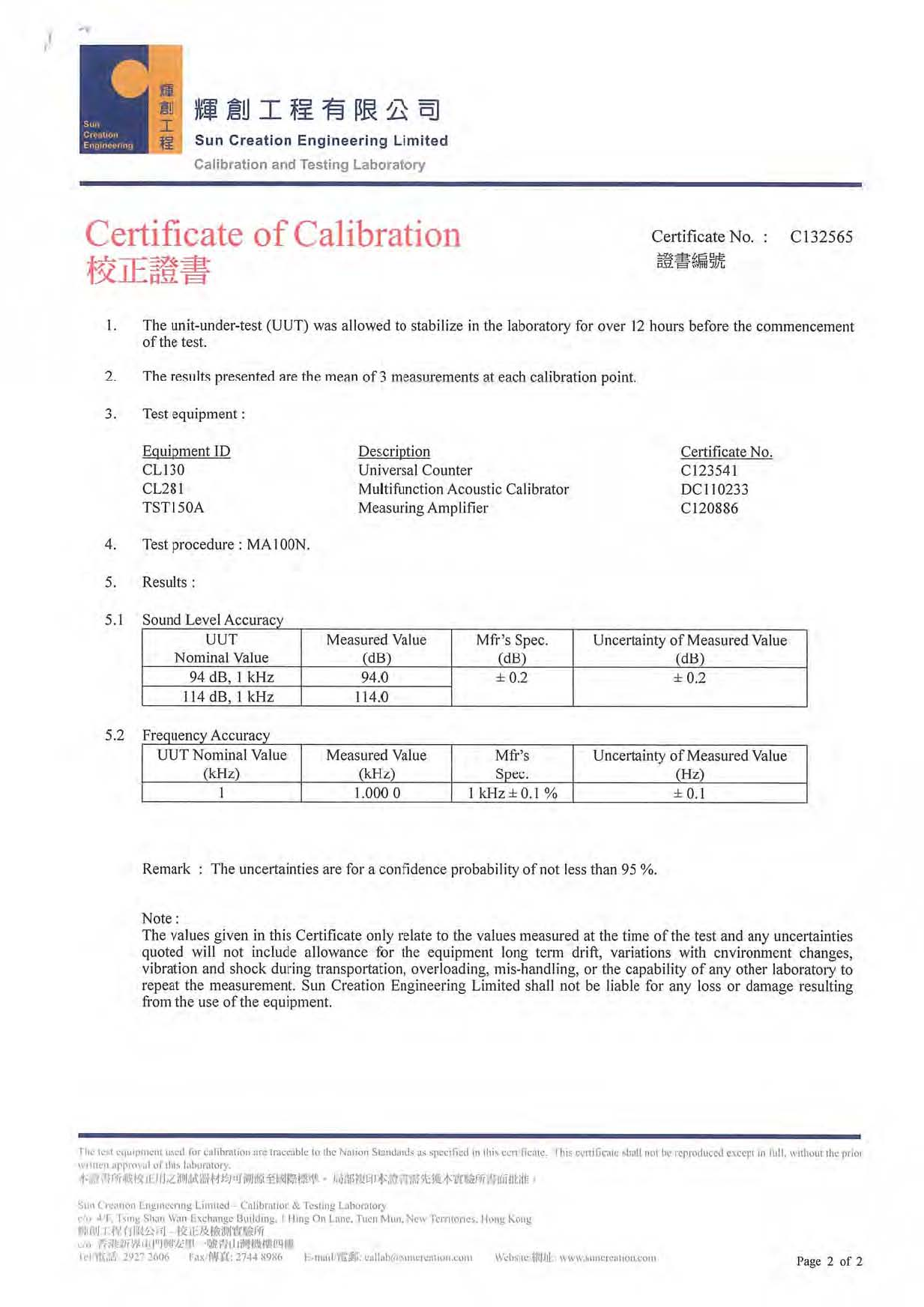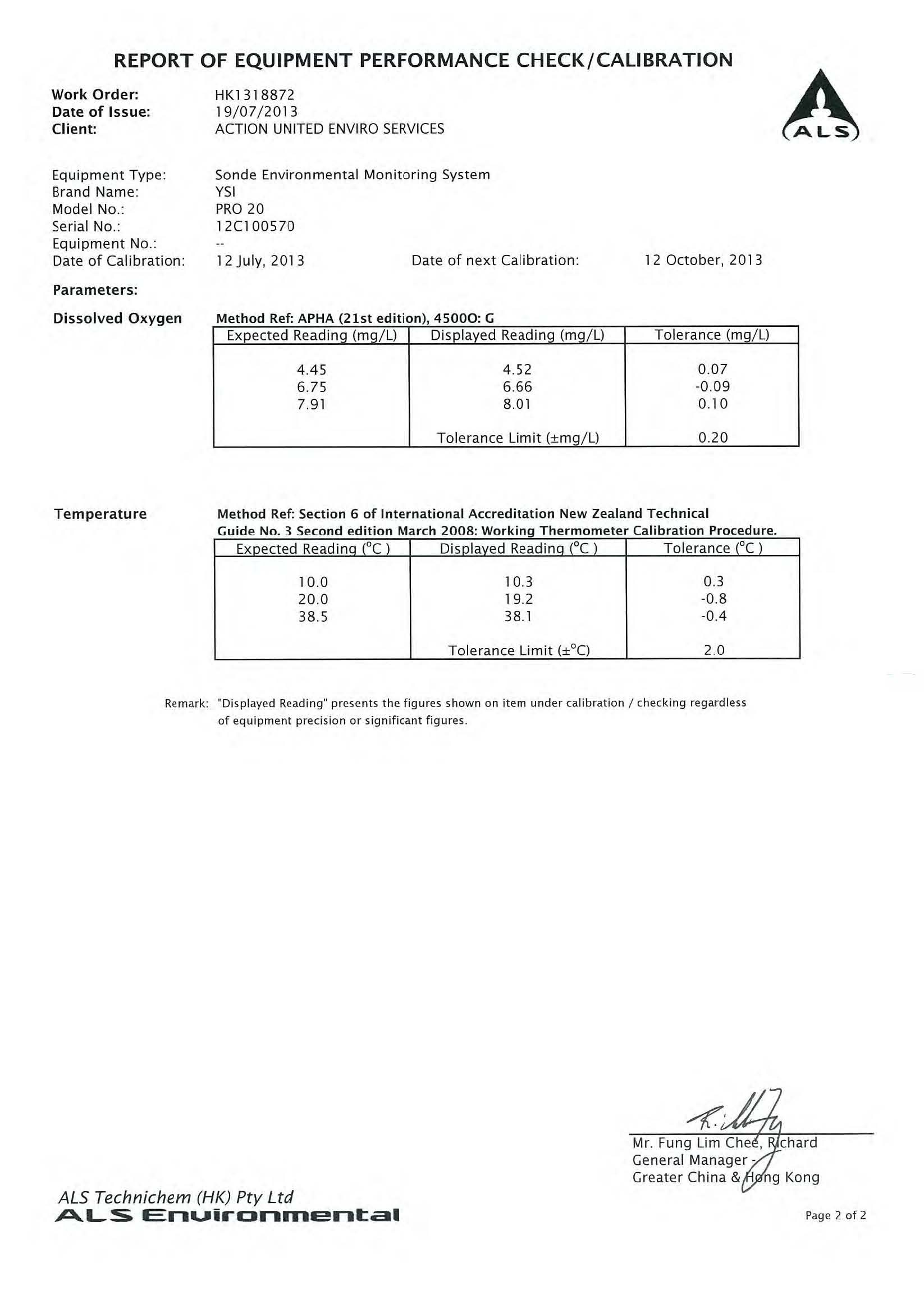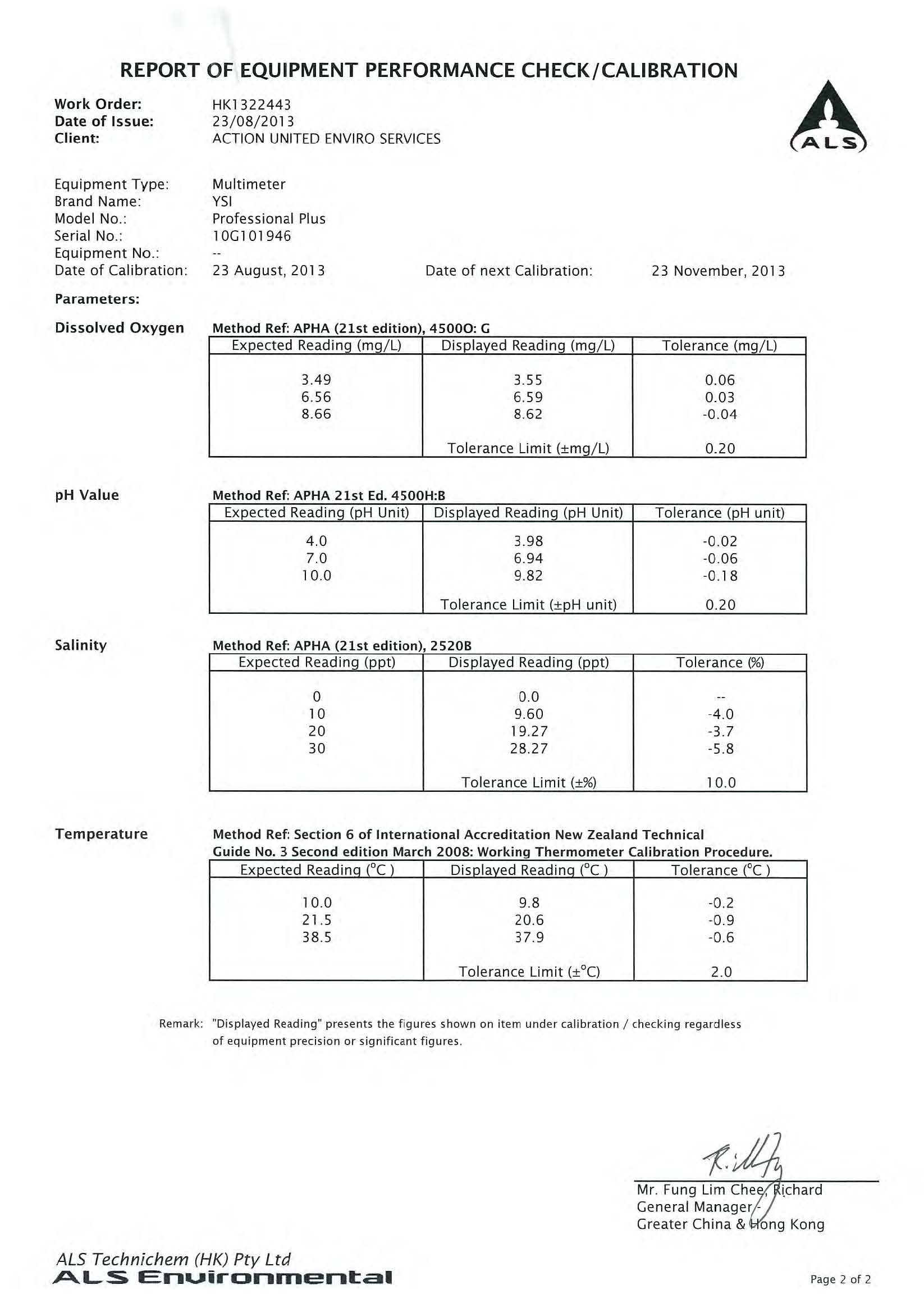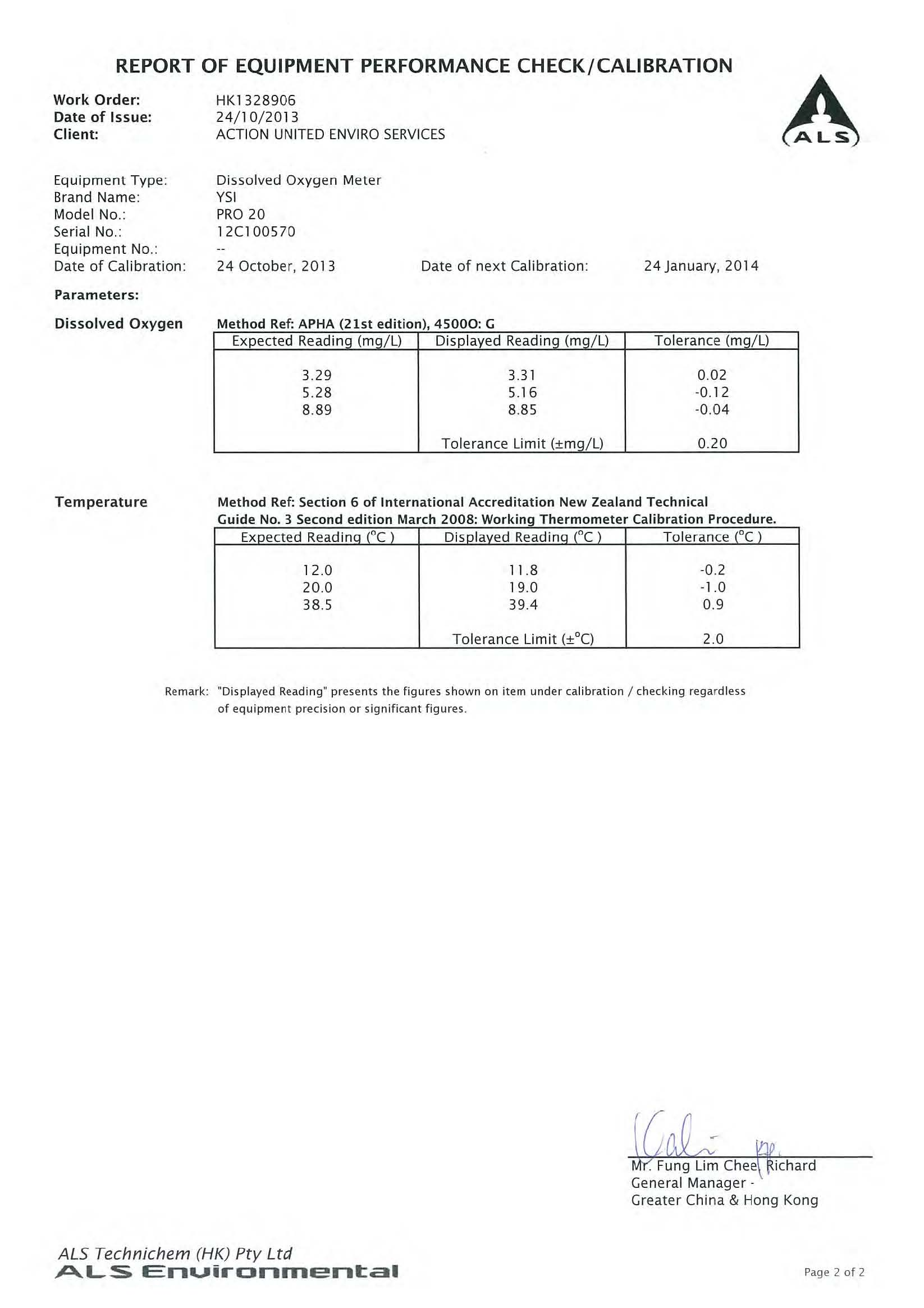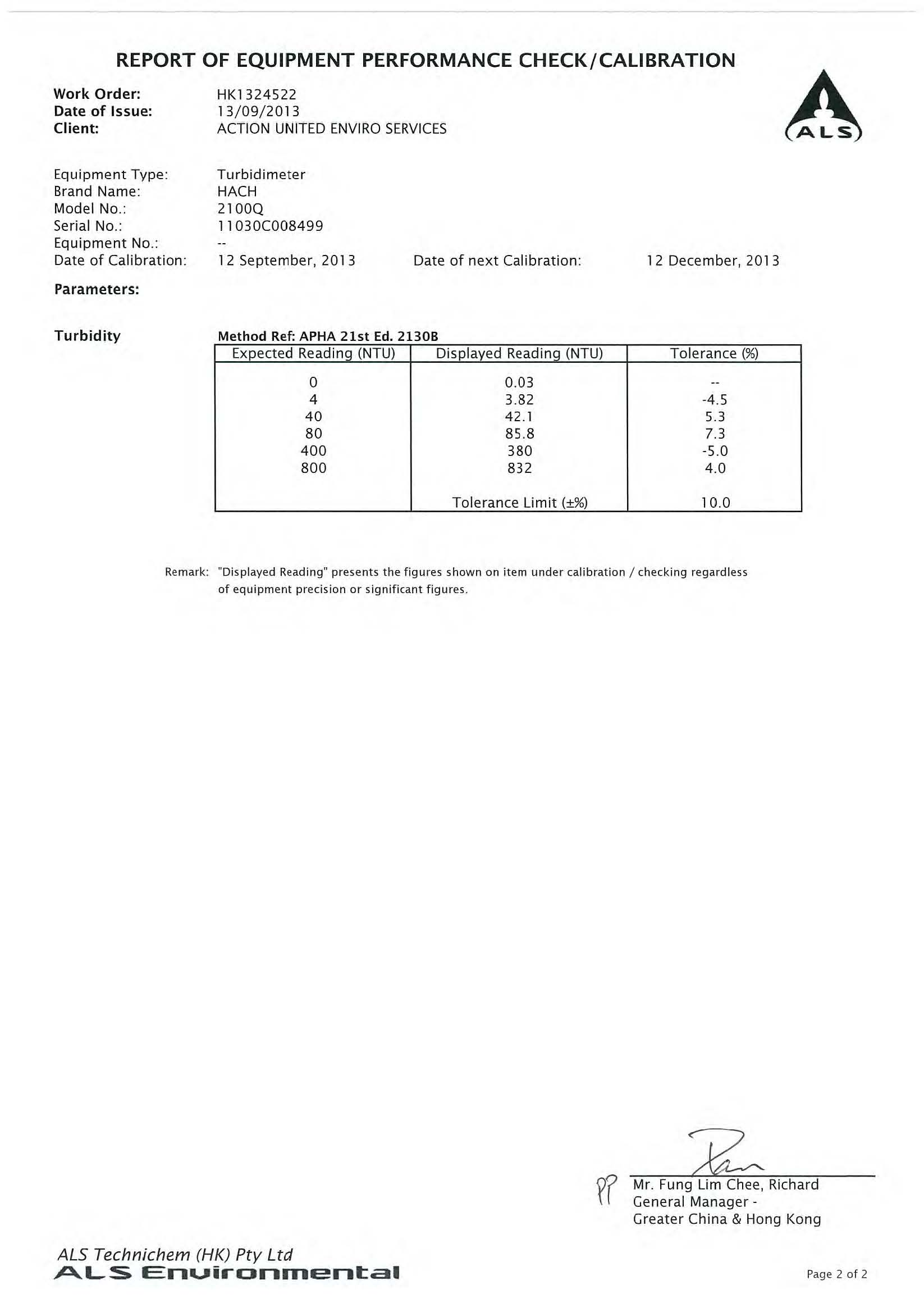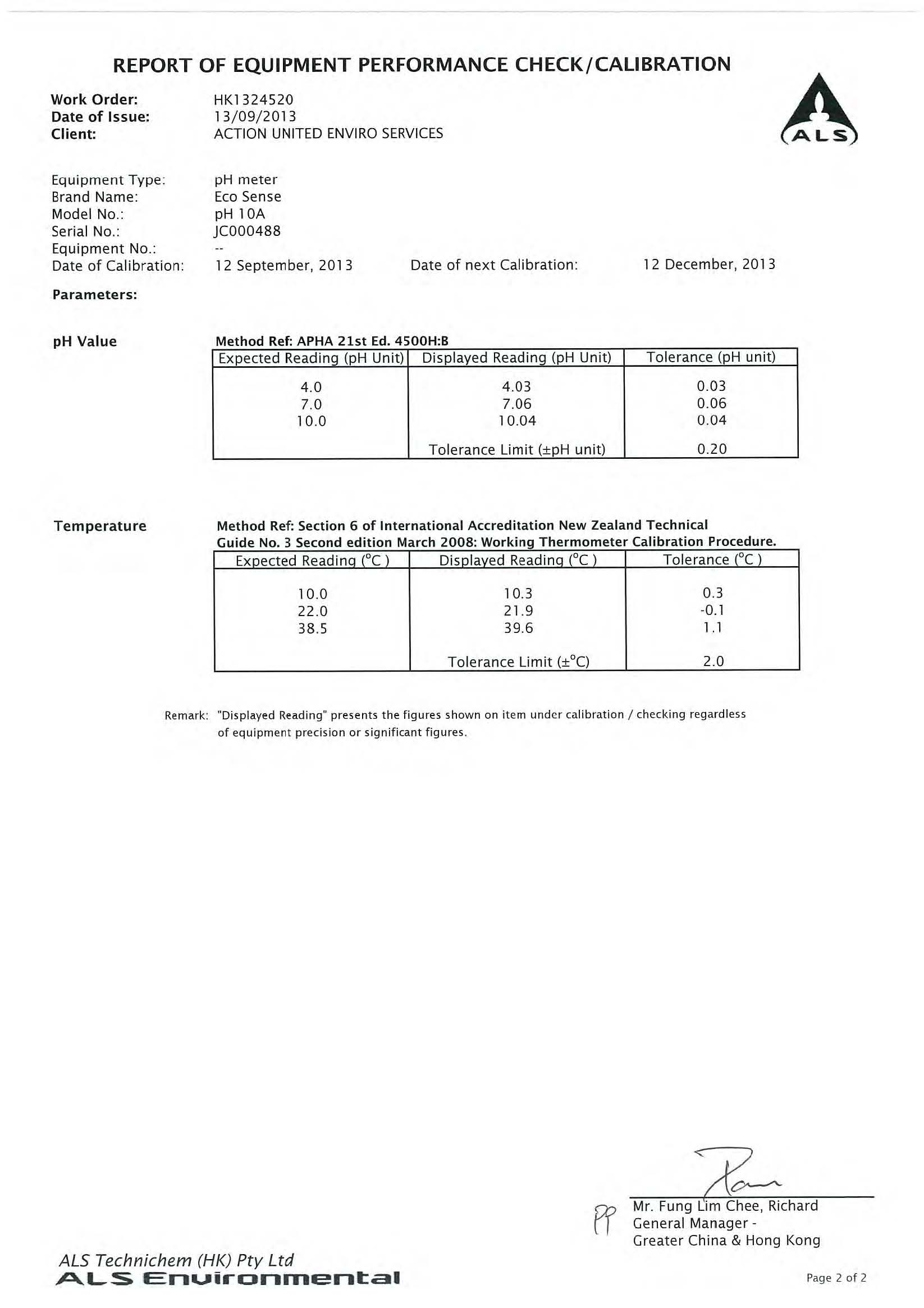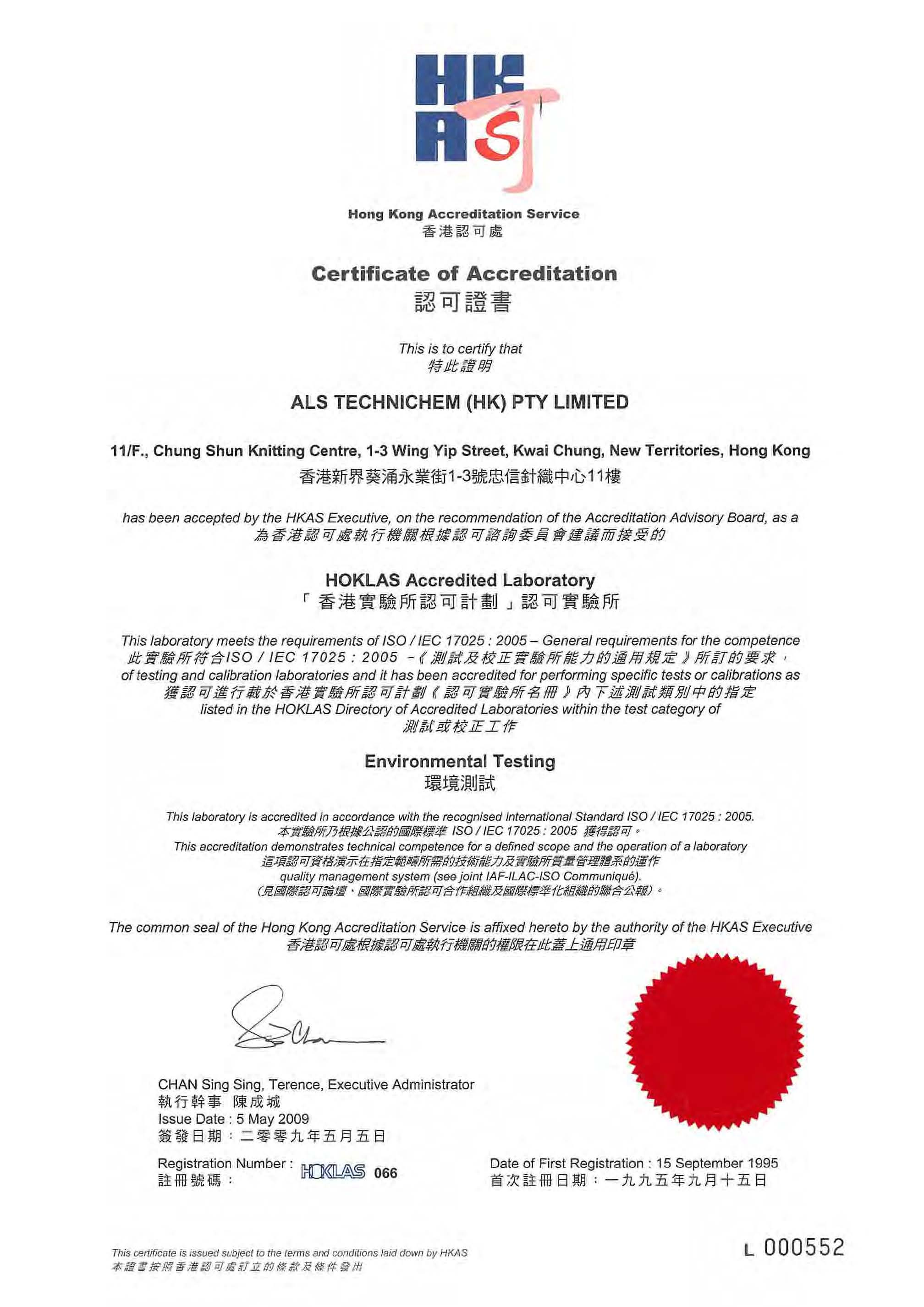##### TSP SAMPLER CALIBRATION CALCULATION SPREADSHEET
Location : Tsung Yuen Ha Village House No. 63 Location ID : AM1 Date of Calibration: 26/8/2013 Next Calibration Date: 26/10/2013 Technician: Ben Tam
CONDITIONS Sea Level Pressure (hPa) 1006.8 Temperature (oC) 28.8 Corrected Pressure (mm Hg) 755.1 Temperature (K) 302
CALIBRATION ORIFICE Make-> TISCH Model-> 5025A Serial # -> 1941 Qstd Slope -> 2.11662 Qstd Intercept -> -0.01714
CALIBRATION
Plate No. H20 (L) (in) H2O (R) (in) H20 (in) Qstd (m3/min) I (chart) IC corrected LINEAR REGRESSION
18 6.8 6.8 13.6 1.734 54 53.15 Slope = 44.9590
13 5.7 5.7 11.4 1.588 45 44.29 Intercept = -26.1666
10 4.7 4.7 9.4 1.443 38 37.40 Corr. coeff. = 0.9960
7 3.4 3.4 6.8 1.228 30 29.53
5 2.5 2.5 5.0 1.054 22 21.65
Calculations : Qstd = 1/m[Sqrt(H20(Pa/Pstd)(Tstd/Ta))-b] IC = I[Sqrt(Pa/Pstd)(Tstd/Ta)] Qstd = standard flow rate IC = corrected chart respones I = actual chart response m = calibrator Qstd slope b = calibrator Qstd intercept Ta = actual temperature during calibration ( deg K ) Pstd = actual pressure during calibration ( mm Hg ) For subsequent calculation of sampler flow: 1/m(( I )[Sqrt(298/Tav)(Pav/760)]-b) m = sampler slope b = sampler intercept I = chart response Tav = daily average temperature Pav = daily average pressure 0.00 10.00 20.00 30.00 40.00 50.00 60.00 0.000 Actual chart response (IC) 0.500 1.000 1.500 2.000 Standard Flow Rate (m3/min) FLOW RATE CHART

Location : Village House near Lin Ma Hang Road Date of Calibration: 26/8/2013 Location ID : AM2 Next Calibration Date: 26/10/2013 Technician: Ben Tam
CONDITIONS Sea Level Pressure (hPa) 1006.8 Corrected Pressure (mm Hg) 755.1 Temperature (oC) 28.8 Temperature (K) 302
CALIBRATION ORIFICE Make-> TISCH Qstd Slope -> 2.11662 Model-> 5025A Qstd Intercept -> -0.01714 Serial # -> 1941
CALIBRATION
Plate No. H20 (L)(in) H2O (R) (in) H20 (in) Qstd (m3/min) I (chart) IC corrected LINEAR REGRESSION
18 13 10 7 5 6.5 5.1 3.6 2 1.6 6.5 5.1 3.6 2 1.6 13.0 10.2 7.2 4.0 3.2 1.695 1.503 1.264 0.944 0.845 55 49 43 35 30 54.13 48.23 42.32 34.45 29.53 Slope = 27.5878 Intercept = 7.2413 Corr. coeff. = 0.9966
Calculations : Qstd = 1/m[Sqrt(H20(Pa/Pstd)(Tstd/Ta))-b] IC = I[Sqrt(Pa/Pstd)(Tstd/Ta)] Qstd = standard flow rate IC = corrected chart respones I = actual chart response m = calibrator Qstd slope b = calibrator Qstd intercept For subsequent calculation of sampler flow: 1/m(( I )[Sqrt(298/Tav)(Pav/760)]-b) m = sampler slope b = sampler intercept I = chart response Tav = daily average temperature Pav = daily average pressure 0 10 20 30 40 50 60 0 0.5 1 1.5 2 Actual chart response (IC) Standard Flow Rate (m3/min) FLOW RATE CHART

Location : Ta Kwu Ling Fire Service Station Location ID : AM3 Date of Calibration: 26/8/2013 Next Calibration Date: 26/10/2013 Technician: Ben Tam
CONDITIONS Sea Level Pressure (hPa) 1006.8 Temperature (oC) 28.8 Corrected Pressure (mm Hg) 755.1 Temperature (K) 302
CALIBRATION ORIFICE Make-> TISCH Model-> 5025A Serial # -> 1941 Qstd Slope -> 2.11662 Qstd Intercept -> -0.01714
CALIBRATION
Plate No. H20 (L)(in) H2O (R) (in) H20 (in) Qstd (m3/min) I (chart) IC corrected LINEAR REGRESSION
18 13 10 7 5 6.2 5 3.9 2.8 1.5 6.2 5 3.9 2.8 1.5 12.4 10.0 7.8 5.6 3.0 1.656 1.488 1.315 1.115 0.819 56 51 45 36 28 55.12 50.20 44.29 35.43 27.56 Slope = 33.9727 Intercept = -0.9187 Corr. coeff. = 0.9965
Calculations : Qstd = 1/m[Sqrt(H20(Pa/Pstd)(Tstd/Ta))-b] IC = I[Sqrt(Pa/Pstd)(Tstd/Ta)] Qstd = standard flow rate IC = corrected chart respones I = actual chart response m = calibrator Qstd slope b = calibrator Qstd intercept For subsequent calculation of sampler flow: 1/m(( I )[Sqrt(298/Tav)(Pav/760)]-b) m = sampler slope b = sampler intercept I = chart response Tav = daily average temperature Pav = daily average pressure 0 10 20 30 40 50 60 0 Actual chart response (IC) 0.5 1 1.5 2 Standard Flow Rate (m3/min) FLOW RATE CHART

Location : Tsung Yuen Ha Village House No. 63 Date of Calibration: Location ID : AM1 Next Calibration Date: Technician: Ben Tam 26/10/2013 26/12/2013
CONDITIONS Sea Level Pressure (hPa) 1018.3 Corrected Pressure (mm Hg) Temperature (oC) 22.0 Temperature (K) 763.725 295
CALIBRATION ORIFICE Make-> TISCH Qstd Slope -> 2.11662 Model-> 5025A Qstd Intercept -> -0.01714 Serial # -> 1941CALIBRATION
Plate No. H20 (L)(in) H2O (R) (in) H20 (in) Qstd (m3/min) I (chart) IC corrected LINEAR REGRESSION
18 6.7 6.7 13.4 1.751 52 52.39 Slope = 41.6678
13 5.7 5.7 11.4 1.615 45 45.34 Intercept = -21.5030
10 4.6 4.6 9.2 1.452 38 38.29 Corr. coeff. = 0.9978
7 3.3 3.3 6.6 1.231 29 29.22
5 2.3 2.3 4.6 1.029 22 22.17
Calculations : Qstd = 1/m[Sqrt(H20(Pa/Pstd)(Tstd/Ta))-b] IC = I[Sqrt(Pa/Pstd)(Tstd/Ta)] Qstd = standard flow rate IC = corrected chart respones I = actual chart response m = calibrator Qstd slope b = calibrator Qstd intercept Ta = actual temperature during calibration ( deg K ) Pstd = actual pressure during calibration ( mm Hg ) For subsequent calculation of sampler flow: 1/m(( I )[Sqrt(298/Tav)(Pav/760)]-b) m = sampler slope b = sampler intercept I = chart response Tav = daily average temperature Pav = daily average pressure 0.00 10.00 20.00 30.00 40.00 50.00 60.00 0.000 0.500 1.000 1.500 Actual chart response (IC) Standard Flow Rate (m3/min) FLOW RATE CHART 2.000

Location : Village House near Lin Ma Hang Road Date of Calibration: 26/10/2013 Location ID : AM2 Next Calibration Date: 26/12/2013 Technician: Ben Tam
CONDITIONS Sea Level Pressure (hPa) 1018.3 Corrected Pressure (mm Hg) 763.725 Temperature (oC) 22.0 Temperature (K) 295
CALIBRATION ORIFICE Make-> TISCH Qstd Slope -> 2.11662 Model-> 5025A Qstd Intercept -> -0.01714 Serial # -> 1941
CALIBRATION
Plate No. H20 (L)(in) H2O (R) (in) H20 (in) Qstd (m3/min) I (chart) IC corrected LINEAR REGRESSION
18 13 10 7 5 6.6 5.1 3.5 2.1 1.4 6.6 5.1 3.5 2.1 1.4 13.2 10.2 7.0 4.2 2.8 1.738 1.528 1.268 0.984 0.805 55 50 43 34 30 55.41 50.38 43.32 34.26 30.23 Slope = 27.6650 Intercept = 7.7418 Corr. coeff. = 0.9988
Calculations : Qstd = 1/m[Sqrt(H20(Pa/Pstd)(Tstd/Ta))-b] IC = I[Sqrt(Pa/Pstd)(Tstd/Ta)] Qstd = standard flow rate IC = corrected chart respones I = actual chart response m = calibrator Qstd slope b = calibrator Qstd intercept For subsequent calculation of sampler flow: 1/m(( I )[Sqrt(298/Tav)(Pav/760)]-b) m = sampler slope b = sampler intercept I = chart response Tav = daily average temperature Pav = daily average pressure 0 10 20 30 40 50 60 0 0.5 1 1.5 2 Actual chart response (IC) Standard Flow Rate (m3/min) FLOW RATE CHART